Home

ARTICLE 62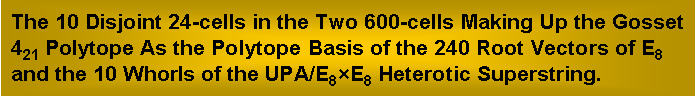by

Stephen M. Phillips

Flat 4, Oakwood House, 117-119 West Hill Road. Bournemouth. Dorset BH2 5PH. England.

Website: http://smphillips.mysite.com

Abstract

 Previous articles by the author have demonstrated that sacred geometries, such as the five 3‑dimensional, regular polyhedrons, embody the root structure of the E8×E8 heterotic superstring symmetry and various structural parameters of the UPA. This is the subatomic particle inside atoms that Theosophists Annie Besant & C.W. Leadbeater claimed to observe with ESP and which the author has interpreted as the subquark state of an E8×E8 heterotic superstring. This article will examine the composition of the six convex regular polychorons — the 4-dimensional counterparts of the Platonic solids — when they are constructed from triangles and tetractyses. The 600-cell (the polychoron with the most faces) is found to embody the superstring structural parameter 840, which has been discussed in many previous articles and shown to characterise various sacred geometries, being the number is the number of turns in half a helical whorl of the UPA. It also embodies the superstring structural parameter 8400, which is the number of turns in an outer or inner half of the UPA. The possession by the 600-cell of these and other structural parameters of holistic systems argues against such properties occurring by chance. The 600-cell emerges uniquely from the analysis as the polychoron counterpart of the inner form of 10 overlapping Trees of Life, just as previous articles have shown that the disdyakis triacontahedron is their polyhedral analogue. The Gosset 421 polytope, whose 240 vertices define the 240 root vectors of the Lie group E8, is a compound of two 600-cells. The construction of the 1200 faces of each 600-cell from triangles requires 8400 geometrical elements. The fact that 16800 geometrical elements are needed to construct the faces of both 600-cells making up the 421 is striking evidence that the UPA with 16800 turns in its 10 helices is an E8×E8 heterotic superstring. The five half-revolutions making up the inner half of the UPA and the five half-revolutions of its outer half would then correspond to the five 24-cells making up each 600‑cell. The 120+120 and 10×24 patterns of vertices in this compound have been previously found in several sacred geometries. It is further evidence of the archetypal nature of the 421 polytope as the polytope representation of the forces and 3-dimensional structure of the UPA, the subquark state of the E8×E8 heterotic superstring. A vertex of each of the 10 24-cells denotes an E8 gauge charge. Its counterpart in the UPA is a set of 70 turns of the whorls.

1

Table 1. Gematria number values of the ten Sephiroth in the four Kabbalistic Worlds.

 SEPHIRAH GODNAME ARCHANGEL ORDER OF ANGELS MUNDANE CHAKRA 1 Kether (Crown)   620 EHYEH (I am)   21 Metatron (Angel of the Presence)   314 Chaioth ha Qadesh (Holy Living Creatures)   833 Rashith ha Gilgalim First Swirlings. (Primum Mobile)   636 2 Chokmah (Wisdom)   73 YAHWEH, YAH (The Lord)   26, 15 Raziel (Herald of the Deity)   248 Auphanim (Wheels)   187 Masloth (The Sphere of the Zodiac)   140 3 Binah (Understanding)   67 ELOHIM (God in multiplicity)   50 Tzaphkiel (Contemplation of God)   311 Aralim (Thrones)   282 Shabathai Rest. (Saturn)   317 Daath (Knowledge)   474 4 Chesed (Mercy)   72 EL (God)   31 Tzadkiel (Benevolence of God)   62 Chasmalim (Shining Ones)   428 Tzadekh Righteousness. (Jupiter)   194 5 Geburah (Severity)   216 ELOHA (The Almighty)   36 Samael (Severity of God)   131 Seraphim (Fiery Serpents)   630 Madim Vehement Strength. (Mars)   95 6 Tiphareth (Beauty)   1081 YAHWEH ELOHIM (God the Creator)   76 Michael (Like unto God)   101 Malachim (Kings)   140 Shemesh The Solar Light. (Sun)   640 7 Netzach (Victory)   148 YAHWEH SABAOTH (Lord of Hosts)   129 Haniel (Grace of God)   97 Tarshishim or Elohim   1260 Nogah Glittering Splendour. (Venus)   64 8 Hod (Glory)   15 ELOHIM SABAOTH (God of Hosts)   153 Raphael (Divine Physician)   311 Beni Elohim (Sons of God)   112 Kokab The Stellar Light. (Mercury)   48 9 Yesod (Foundation)   80 SHADDAI EL CHAI (Almighty Living God)   49, 363 Gabriel (Strong Man of God)   246 Cherubim (The Strong)   272 Levanah The Lunar Flame. (Moon)   87 10 Malkuth (Kingdom)   496 ADONAI MELEKH (The Lord and King)   65, 155 Sandalphon (Manifest Messiah)   280 Ashim (Souls of Fire)   351 Cholem Yesodoth The Breaker of the Foundations. The Elements. (Earth)   168

 The Sephiroth exist in the four Worlds of Atziluth, Beriah, Yetzirah and Assiyah. Corresponding to them are the Godnames, Archangels, Orders of Angels and Mundane Chakras (their physical manifestation). This table gives their number values obtained by the ancient practice of gematria, wherein a number is assigned to each letter of the alphabet, thereby giving a number value to a word that is the sum of the numbers of its letters. Numbers from the table are written in boldface in the article.

2

1. The 6 polychorons

A polytope is a geometric object with straight sides that exists in any number of dimensions. In two dimensions a polytope is a polygon and in three dimensions it is a polyhedron. If a polytope contains all the points on a segment joining any pair of points in it, the polytope is convex. An n-polytope is an n-dimensional polytope. An n-polytope is regular if any set consisting of a vertex, an edge containing it, a 2-dimensional face containing the edge, and so on up to n−1 dimensions, can be mapped to any other such set by a symmetry of the polytope. The regular polygons form an infinite class of 2-polytopes. There are five regular convex polyhedra, or 3-polytopes, known as the Platonic solids. There exist six types of convex, regular 4-polytopes known as the polychorons, but only three types of n-polytopes when n5.

The elements of a polytope are its vertices, edges, faces, cells (polyhedra) and so on. These elements can be represented by the following Schäfli symbols:

regular p-gon: {p}.

regular polyhedron with q regular p-gon faces meeting at a vertex: {p,q}.

regular 4-polytope with r {p,q} regular polyhedral cells around each edge: {p,q,r}.

and so on. The dual of the {p,q} polyhedron is the {q,p} polyhedron, i.e., vertices and faces are interchanged. It is self-dual when p = q. The dual of the 4-polytope {p,q,r} has the Schäfli symbol {r,q,p}. A 4-polytope is self-dual if it is identical to its dual. This means that a self-dual 4-polytope has the Schäfli symbol {p,q,p}. The Schäfli symbols of the five Platonic solids are:

tetrahedron: {3,3}.

octahedron: {3,4}.

cube: {4,3}.

icosahedron: {3,5}.

dodecahedron: {5,3}.

If a vertex of a polyhedron is sliced off, the surface created is bounded by a polygon called the vertex figure. A regular polyhedron has always a regular polygon as its vertex figure. A {p,q} regular polyhedron has the regular vertex figure {q}. The vertex figure of a polychoron is a polyhedron, seen by the arrangement of neighbouring vertices around a given vertex. For regular polychora with the Schäfli symbol {p,q,r}, this vertex figure is a {q,r} regular polyhedron. The vertex figure for an n-polytope is an (n1)-polytope. An edge figure for a polychoron is a polygon, seen by the arrangement of faces around an edge. For regular {p,q,r} polychora, this edge figure will always be a regular polygon {r}. Regular polychora with Schäfli symbol {p,q,r} have r polyhedral cells of type {p,q} around each edge, faces of type {p}, edge figures {r} and vertex figures {q,r}.

Table 2 lists the geometrical elements making up the six convex, regular polychorons:

Table 2. The geometrical composition of the regular polychorons.

 Polychoron Schäffli symbol {p,q,r} Vertices V {q,r} Edges E {r} Faces F {p} Cells C {p,q} Dual 5-cell {3,3,3} 5 {3,3} 10 {3} 10 {3} 5 {3,3} Self 8-cell {4,3,3} 16 {3,3} 32 {3} 24 {4} 8 {4,3} 16-cell 16-cell {3,3,4} 8 {3,4} 24 {4} 32 {3} 16 {3,3} 8-cell 24-cell {3,4,3} 24 {4,3} 96 {3} 96 {3} 24 {3,4} Self 120-cell {5,3,3} 600 {3,3} 1200 {3} 720 {5} 120 {5,3} 600-cell 600-cell {3,3,5} 120 {3,5} 720 {5} 1200 {3} 600 {3,3} 120-cell

(The Schäfli symbols of the vertex and edge figures are given for each vertex and edge count of a polychoron).

An n-cell is a polychoron with n polyhedral cells:

3

• The 5-cell (also called the 4-simplex, or pentachoron) with five vertices, 10 edges, 10 triangular faces & five tetrahedral cells is the 4-dimensional counterpart of the tetrahedron, both being self-dual;
• The 8-cell (also called the 4-cube, or tesseract) with 16 vertices, 32 edges, 24 square faces & eight cubic cells (four around each of its 32 edges) is the 4-dimensional counterpart of the cube;
• The 16-cell with eight vertices, 24 edges, 32 triangular faces & 16 tetrahedral cells (four meeting at each of 24 edges) is the 4-dimensional counterpart of the octahedron;
• The 24-cell, which was first discovered by mathematician Ludwig Schäfli in 1852, has no Platonic counterpart and — like the 5-cell — is self-dual. It has 24 vertices, 96 cells, 96 triangular faces & 24 octahedral cells, three being around each of its 96 edges.
• The 120-cell with 600 vertices, 1200 edges, 720 pentagonal faces & 120 dodecahedral cells is the 4-dimensional counterpart of the dodecahedron with 12 pentagonal faces. It has three dodecahedra around each of its edges, just as the dodecahedron has three pentagonal faces meeting at each of its 20 vertices;
• The 600-cell with 120 vertices, 720 edges, 1200 triangular faces & 600 tetrahedral cells is the 4‑dimensional counterpart of the icosahedron with 20 triangular faces. It has five tetrahedra meeting at every one of its edges, just as the icosahedron has three triangles meeting at each of its 12 vertices.

2. Geometrical composition of the polychorons

A polychoron has V vertices, E edges, F faces & C cells, where

V  E + F − C = 0.

As regular m-gons (m = 3, 4 or 5), the lattermost are divided into their m sectors. Joining its vertices to its centre generates E internal triangles. Treating them as Type A triangles generates (E+1) new corners and (V+3E) new sides of 3E new triangles.

Faces

Number of corners of mF triangles in F faces  C = V + F.

Number of sides of mF triangles  S = E + mF.

Number of triangles  T = mF.

Number of corners, sides & triangles  N = C + S + T = V + E + (2m+1)F.

Interior

Number of corners of 3E triangles  C = 1 + E

Number of sides of 3E triangles = S = V + 3E.

Number of triangles = T = 3E.

Faces + interior

Number of corners, sides & triangles = N = C + S + T = 1 + V + 7E.

Total number of corners  c = C + C = 1 + V + E + F.

Total number of sides  s = S + S = V + 4E + mF.

Total number of triangles  t = T + T = 3E + mF.

Total number of corners, sides & triangles  n = N + N = 1 + 2V + 8E + (2m+1)F.

Table 3 lists the geometrical composition of the 6 polychorons:

Table 3. Geometrical composition of faces and interiors of the 6 polychorons.

 Polychoron Faces Interior Total C S T N C′ S′ T′ N′ c s t n 5-cell 15 40 30 85 1+10 35 30 1+75 1+25=26 75 60 1+160 8-cell 40 128 96 264 1+32 112 96 1+240 1+72=73 240 192 1+504 16-cell 40 120 96 256 1+24 80 72 1+176 1+64=65 200 168 1+432 24-cell 120 384 288 792 1+96 312 288 1+696 1+216=217 696 576 1+1488 120-cell 1320 4800 3600 9720 1+1200 4200 3600 1+9000 1+2520=2521 9000 7200 1+18720 600-cell 1320 4320 3600 9240 1+720 2280 2160 1+5160 1+2040=2041 6600 5760 1+14400

(“1” denotes the centre of the polychoron).

4

5-cell

1) The number value 15 of YAH (YH), the shortened Godname of Chokmah, is the number of points needed to construct the faces of the 4-d tetrahedron from triangles. The value 5 of H is the number of vertices and the value 10 of Y is the additional number of corners of the 30 triangles in its faces. The number value 26 of YAHWEH, the full Godname of this Sephirah, is the total number of corners of the 60 triangles making up the 5-cell. The number value 21 of EHYEH, the Godname of Kether, is the number of points in it other than vertices. The total number of their corners & sides is (26+75=101). This is the 26th prime number. 100 (=13+23+33+43) corners & sides surround the centre of the 5-cell.

2) The 30 triangles in the 10 faces comprise (15+40=55) corners & sides, where

 1 2 3 55 = 4 5 6 7 8 9 10

is the 10th triangular number. The faces comprise 85 geometrical elements, where

85 = 40 + 41 + 42 + 43,

of which 15 are points and 70 are lines & triangles. Compare these properties with fact that the 2nd-order tetractys contains 85 yods, of which 15 are corners of the 10 1st-order tetractyses and 70 are hexagonal yods (Fig. 1):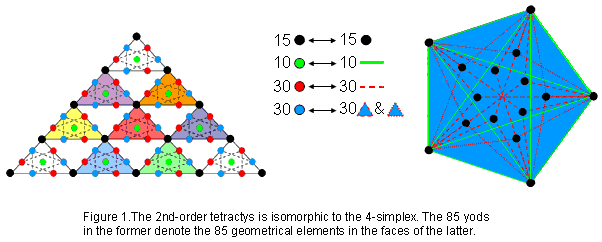The four rows of a 1st-order tetractys traditionally symbolise the sequence:

0-simplex1-simplex→2-simplex→3-simplex.

We now see that the 2nd-order tetractys symbolises the 4-simplex, the 15 black corners of the 10 1st-order tetractyses symbolizing the 15 points in 4-d space that are the corners of the 30 constituent triangles in its faces, the 10 green hexagonal yods at the centres of the 10 1st-order tetractyses denoting its 10 edges and the remaining 60 hexagonal yods (30 red, 30 blue) denoting the 30 triangular sectors of its 10 faces and their 30 remaining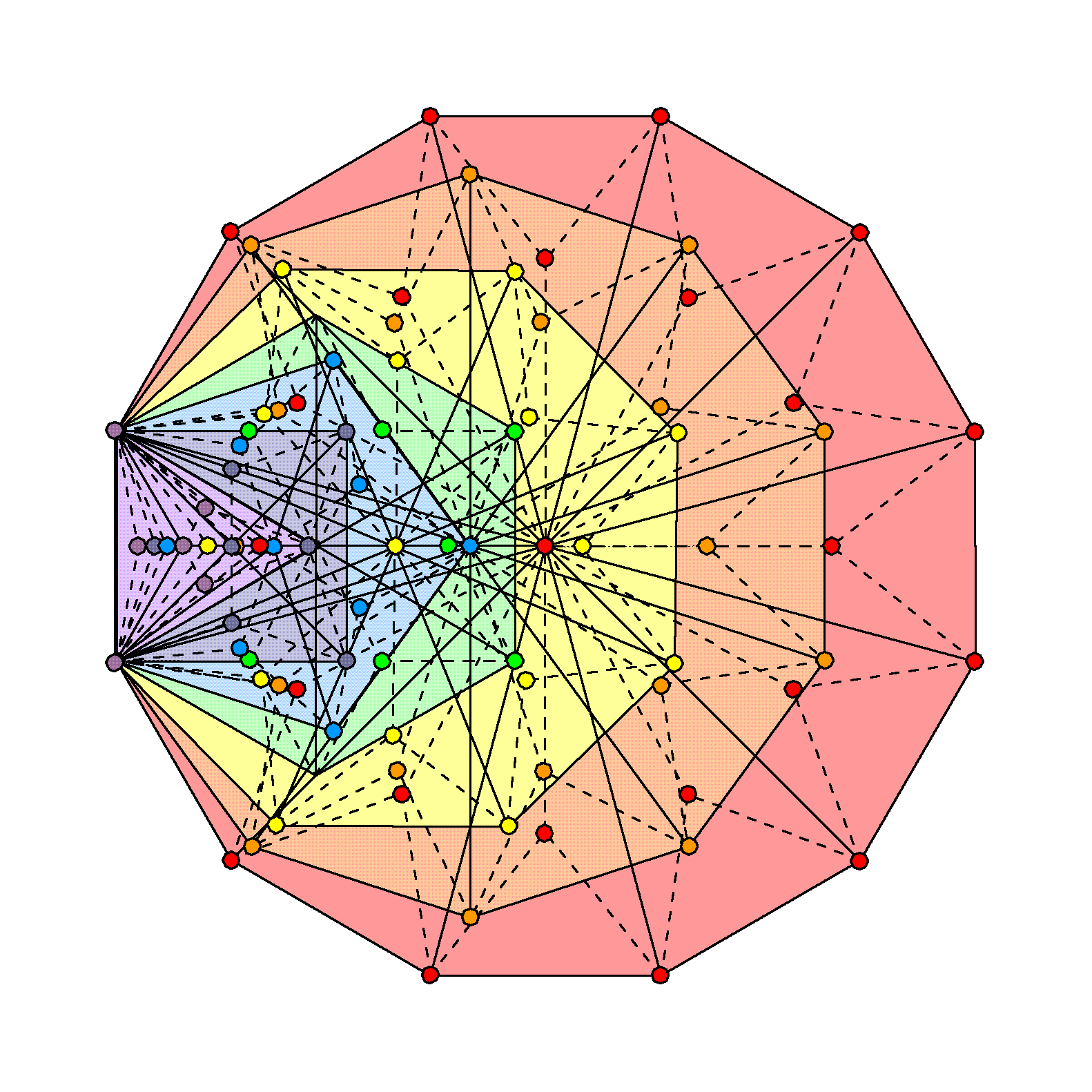Figure 2. The 141 triangular sectors of the 7 enfolded Type B polygons have 85 corners unshared with the outer Tree of Life.

sides. Just as the tetrahedron (3-simplex) is the starting point in the build-up of the five regular polyhedra in 3-dimensional space, so the 4-simplex as the simplest polychoron commences the build-up of the six regular polychora in 4‑dimensional space. The Pythagorean representation of holistic systems — the 2nd-order tetractys — represents the 4-simplex. The counterpart of this in the inner Tree of Life are the 85 corners of the 141 triangles making up the 47 sectors of the seven enfolded Type B polygons that are intrinsic to them because none are corners of triangles belonging to the outer Tree of Life (Fig. 2). Notice that no coloured corners are depicted at the top, centre & bottom of the hexagon because these points are located at Chokmah, Chesed & Netzach. It might be thought that the seven enfolded polygons with 88 corners of their 141 triangles could, alternatively, embody the number 85 as the 85 corners outside the root edge that are

5

intrinsic to each set of polygons enfolded in successive Trees of Life. However, this cannot be the ‘right’ embodiment because, in the former scheme, the embodiment of the superstring structural parameter 168 emerges naturally as the (84+84=168) corners of the 282 triangles composing the (47+47=94) sectors of the (7+7) enfolded polygons that are not corners of triangles in the outer Tree of Life, whereas in the latter scheme, the (7n+7n) polygons enfolded in n Trees of Life have 282n triangles with (170n+2) corners, but there is no reason for removing just one corner from each set of seven polygons so as to leave the required set of (168n+2) corners. The property that the (70+70) polygons enfolded in the 10‑tree should have 2820 triangles with 1680 corners (the representation of the 1680 turns of each helical whorl of the 10-dimensional UPA), leads, therefore, to a unique geometrical interpretation of the number 85 as a holistic parameter. Its presence in the 4-simplex, shaping its faces, indicates that the Pythagorean archetypal symbol of the tetractys extends beyond 3-dimensional space into four dimensions through its next higher-order version.

3) (855=80) geometrical elements are needed to create the faces of the 5-simplex from five equidistant points in 4-dimensional space. This is the number of Yesod, the penultimate Sephirah. Its interior has 76 geometrical elements, where 76 is the number value of YAHWEH ELOHIM, the Godname of Tiphareth. 155 geometrical elements other than vertices surround its centre, with 50 points & lines in its faces. This shows how ELOHIM, the Godname of Binah with number value 50, and ADONAI MELEKH, the Godname of Malkuth with number value 155, prescribes the geometrical composition of the 5-simplex. It includes 65 lines other than edges, where 65 is the number value of ADONAI.

There are 30 vertices, edges, triangular faces & tetrahedral cells, where

30 = 12 + 22 + 32 + 42.

8-cell
1) The 96 triangular sectors of its 24 square faces are formed by 40 points and 128 straight lines, i.e., 168 points & lines. This is how the tesseract embodies the superstring structural parameter 168. 264 geometrical elements make up its faces. This is the number of yods in the seven enfolded polygons that constitute each half of the inner Tree of Life (Fig. 3).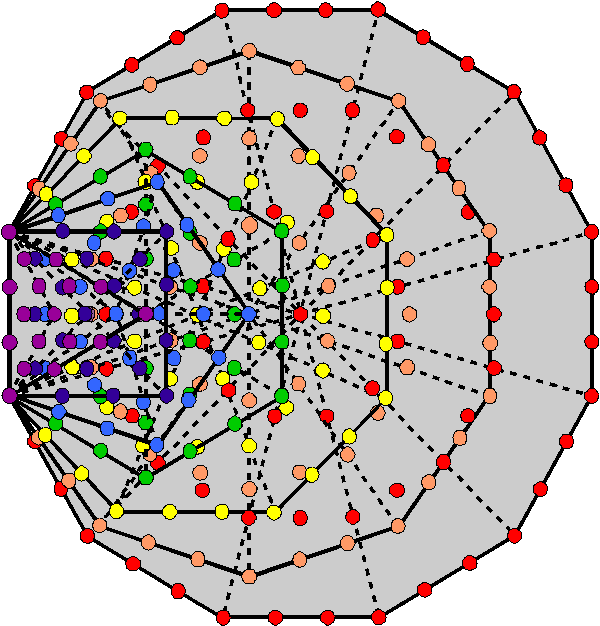Figure 3. The 47 sectors of the 7 enfolded polygons of the inner Tree of Life have 264 yods when the sectors are tetractyses.

2) 240 geometrical elements inside the 8-cell surround its centre. They comprise 128 corners & triangles and 112 sides. The 240 sides comprise 128 sides in its faces and 112 sides in its interior.

3) The 192 triangles in the 8-cell have 73 corners, where 73 is the number value of Chokmah.

4) There are 72 vertices, edges, square faces & cubic cells. Including the eight cubic cells, the 8-cell has 80 vertices, edges, faces & cells, where 80 is the number of Yesod.

16-cell

1) Its 32 triangular faces contain 256 geometrical elements, where 256 = 44, showing how the Tetrad expresses its geometrical composition. They have 65 corners of 168 triangles, where 65 is the number of ADONAI, the Godname of Malkuth, and 168 is the number of Cholem Yesodoth, the Mundane Chakra of this Sephirah. 432 geometrical elements surround its centre, demonstrating the role of the integers 1, 2, 3 & 4 symbolised by the tetractys in expressing properties of regular polyhedra and polychorons.

2) There are 80 vertices, edges, triangular faces & tetrahedral cells.

24-cell

1) The 96 triangular faces consist of 288 triangles with 120 corners and 384 sides, where 120 is the sum of the first 10 odd integers after 1 and 288 = 11 + 22 + 33 + 44. It has 288 internal triangles, so that 576 triangles are present, where 576 = 242 = 12×22×32×42. Compare these properties with the following facts:

• 288 yods line the boundaries of the (7+7) separate, Type A polygons;
• 288 yods surround the centres of the 7 separate, Type A polygons;
• 120 yods line the sides of each set of seven enfolded polygons;
• 384 corners, sides & triangles and (288+288=576) yods surround the centres of the (7+7) separate polygons.

6

As the 600-cell is the convex hull of five disjoint 24-cells, this display of holistic parameters in the 24-cell suggests that the former polychoron, too, is holistic in nature. Later discussion of the 600-cell will confirm this.

2) 216 points (=63) surround the centre of the 24-cell with Type A faces and internal triangles. This is the number of Geburah, the sixth Sephirah.
3) 1488 geometrical elements surround the centre. They include 24 vertices and 96 edges, i.e., 120 points & lines, so that (1488−120=1368) new geometrical elements are needed to construct the 24-cell. As the (7+7) enfolded Type B polygons contain 1370 yods (Fig. 4),Figure 4. The (7+7) enfolded Type B polygons have 1370 yods.

of which two yods (topmost corners of the two hexagons) are shared with the two similar sets of polygons enfolded in the next higher Tree, 1368 yods are intrinsic to each set of polygons.

4) There are 240 vertices, edges, triangular faces & octahedral cells.

120-cell

1) The 18720 geometrical elements surrounding its centre include 720 centres of its pentagonal faces and 1200 centres of the internal triangles formed by its 1200 edges, i.e. 1920 points. The (187201920=16800) geometrical elements other than these centres include (9720720=9000=90×10×10) elements in faces and (4200+3600=7800=78×10×10) internal sides & triangles. This is remarkable, because the number value 168 of Cholem Yesodoth, the Mundane Chakra of Malkuth, is the sum of the number 78 of Cholem and the number 90 of Yesodoth. The 120-cell is the only polychoron that is built from a number of geometrical elements that exceeds 16800, so that it is the only one that could embody this superstring structural parameter, namely, the number of turns in the 10 helices of the UPA/superstring. It is implausible that both these properties could arise by chance. Moreover, the algebraic expression for the population of geometrical elements other than centres of faces or internal triangles formed by edges is

2V + 7E + 2mF = (2V+2mF) + 7E

= (1200+7200=8400) + 7×1200 = 8400 + 8400 = 16800

for the 120-cell. As 2V = E for this polychoron, the expression may be rearranged in this case as (E+2mF) + (6E+2V). It enables ready interpretation, for “(E+2mF)” is the number (8400) of geometrical elements in the faces other than points and “(6E+2V)” is the number (8400) of vertices & internal geometrical elements other than corners of triangles. The 120-cell therefore embodies the 16800 turns of the 10 whorls of the UPA as the 8400 turns in their 2½ outer revolutions and the 8400 turns in their 2½ inner revolutions.

2) The general algebraic expression discussed above may be rewritten:

6E + (2V+E) + 2mF

7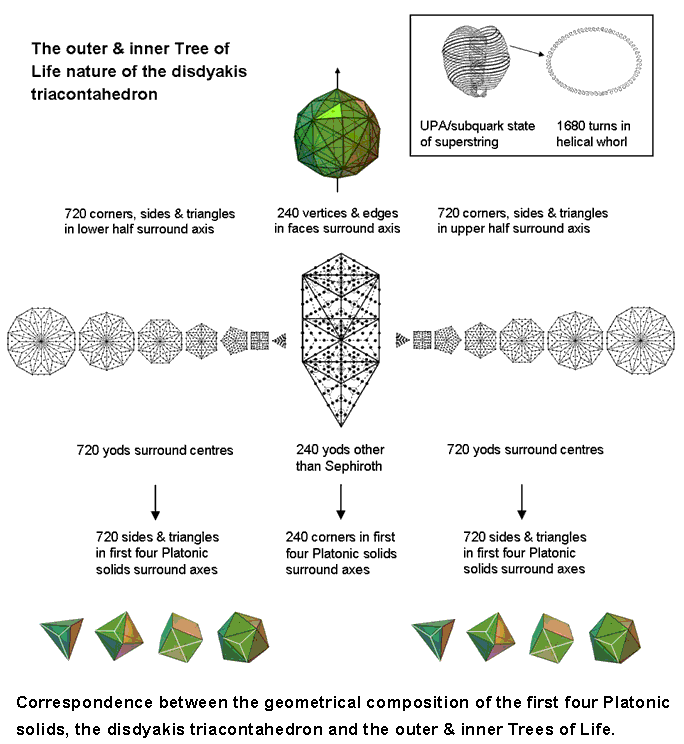Figure 5. Correspondences between the geometrical composition of the first four Platonic solids, the disdyakis triacontahedron and the outer & inner Trees of Life.

For the 120-cell, “6E” is the number (7200) of internal lines & triangles formed by the 1200 edges, “(2V+E)” is the number (2400) of vertices, edges & internal sides joining vertices to the centre of the polychoron and “2mF” is the number of lines & triangles generated by dividing the faces into their sectors. Therefore, 16800 = 7200 + 2400 + 7200, where the first and third numbers refer to elements created by turning, respectively, internal triangles and faces into Type A triangles and where the second term refers to the number of external & internal points & lines before this transformation. Apart from a Pythagorean factor of 10, this sum has its counterparts in the outer and inner forms of the 1-tree (the lowest of any set of Trees), the first four Platonic solids and the disdyakis triacontahedron (Fig. 5).

Outer & inner forms of the 1-tree

When its 19 triangles are Type A, the 1-tree contains 240 yods other than their 11 corners. Each set of the seven separate Type B polygons making up its inner form has 720 yods surrounding their centres.

1st 4 Platonic solids
When the F faces of a Platonic solid with E edges are divided into their n sectors and their vertices & face centres are joined to the centre of each polyhedron, creating internal triangles that are treated as Type A, the number of corners, sides & triangles that surround an axis passing through two opposite vertices = 10E + 9mF. The tetrahedron (E = 6; mF = 12) has 168 such geometrical elements, both the octahedron (E = 12; mF = 24) & cube (E = 12; mF = 24) have 336 elements and the icosahedron (E =

8

30; mF = 60) has 840 elements. The axes of the first four Platonic solids, which the ancient Greeks believed were the shapes of the particles of the elements of Fire, Water, Earth & Air, are surrounded by 1680 geometrical elements (840 in the tetrahedron, octahedron & cube and 840 in the icosahedron). They comprise 240 corners and 720 sides & triangles in each set of their halves.

Disdyakis triacontahedron

When the 62 vertices of the disdyakis triacontahedron with 180 edges are joined to its centre and the resulting 180 internal triangles turned into Type A triangles, 1680 corners, sides & triangles in its faces and interior surround an axis passing through any two diametrically opposite vertices. They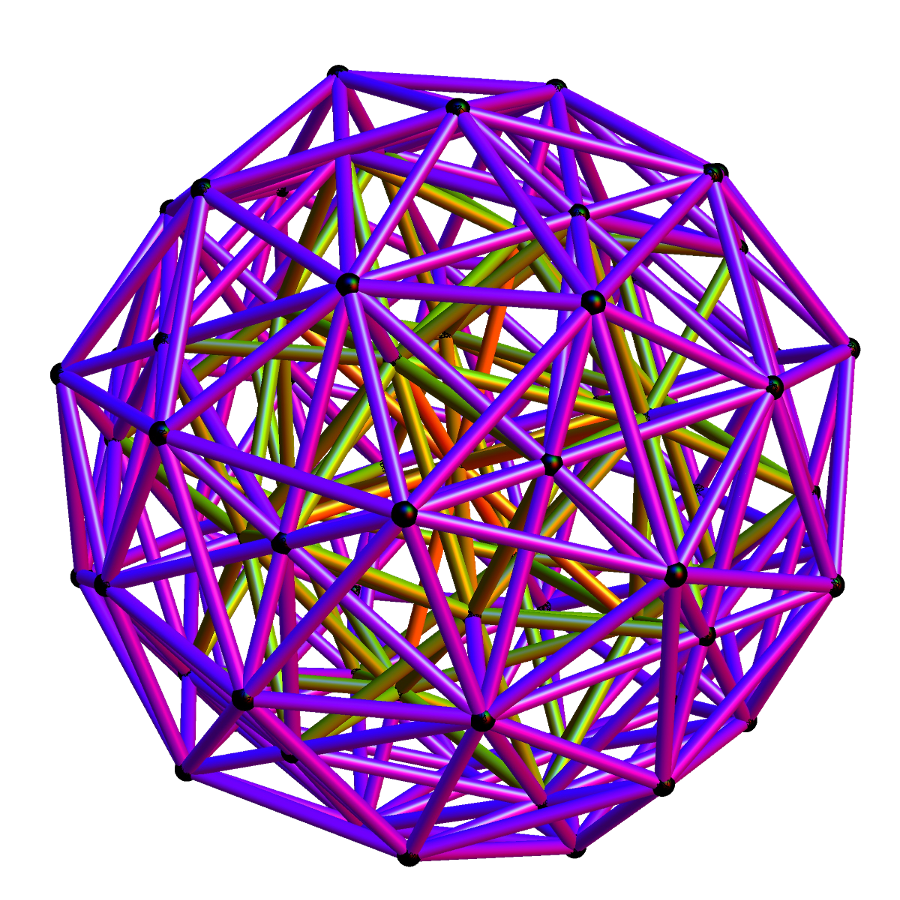Figure 6. 3-dimensional projection of the 600-cell. (Credit: uploaded to Wikipedia by Jgmoxness).

comprise (60+180=240) vertices & edges and 1440 corners (720 in each half of the polyhedron).

3) There are 2640 (=264×10) vertices, edges, pentagonal faces & dodecahedral cells, where 264 is the number of yods in the seven enfolded Type A polygons (see Figure 3).

4)

 The 120-cell

600-cell

1) Figure 6 shows a three-dimensional projection of the 600-cell. Surrounding the centre of the 600-cell are 14400 (=1202) geometrical elements, where

 3 5 7 120 = 7 9 11 13 15 17 19

Is the sum of the first 10 odd integers after 1. This beautiful property shows how the Decad determines the geometrical composition of the 600-cell. As 120 = 5!, it is also determined by the Tetrad because 14400 is the square of the factorial of the fourth integer after 1. The number of points surrounding its centre = 2040 = 24×85 = 1×2×3×4(40+41+42+43), showing that it, too, is expressed by the Tetrad. Of these, 120 (= 23 + 24 + 25 + 26) are vertices, leaving 1920 points, where 1920 = 64×30 = 26(21+22+23+24) = 27 + 28 + 29 + 210. The number of triangles = 5760 = 10×242 = (1+2+3+4)×12×22×32×42. The number of triangles in its 600 faces = 3600 = 4×900 = 4×302 = 4×(12+22+32+42)2. The 9240 points, lines & triangles in its faces include (120+720=840) vertices & edges and 8400 new geometrical elements created by its construction from triangles. This is easily seen from the fact that inside each of the 1200 faces as Type A triangles are a corner & three sides of three triangles, i.e., seven geometrical elements, so that (1200×7=8400) new elements are generated when the faces become Type A triangles. 840 = 84×10 = (12+32+52+72)×(1+2+3+4) and 8400 = (12+32+52+72)×(13 +23+33+4 3). As 120 =5! and 720 = 6!, 840 = 5! + 6!. As each edge is the side of an internal Type A triangle, the 5160 internal geometrical elements surrounding the centre comprise 5! sides terminating on vertices and (7×720=7!) geometrical elements generated by the edges, i.e., 5160 = 5! + 7!.

The possession by the 600-cell of 840 vertices & edges and 10 times as many geometrical elements in its faces when they are Type A polygons is an unambiguous indication of the relevance of this polychoron to superstring theory. This is because both the number 840 and the number 8400 are superstring structural parameters, being, respectively, the number of turns in an outer or inner half of, respectively, a single helical whorl and all 10 whorls of the UPA examined with micro-psi by Besant & Leadbeater. The 120-cell embodies the superstring 16800, but only as a subset of its geometrical elements, namely, the 16800 elements surrounding its centre other than centres of Type A faces and Type A internal triangles. Is, however, the display of superstring structural parameters like 168, 840, 1680, etc unique to the 600‑cell? The number of geometrical elements other than vertices and edges needed to divide each of the F faces of a polychoron into m sectors is (2m+1)F. Table 4 shows these values for the six polychorons. Also displayed is the number (C+S) of the corners & sides of the triangles in their faces.

9

Table 4. Number of geometrical elements other than vertices & edges generating Type A faces.

 Polychoron Vertices V Edges E Faces F V+E m (2m+1)F C+S 5-cell 5 10 10 15 3 70 70 8-cell 16 32 24 48 4 216 168 16-cell 8 24 32 32 3 224 160 24-cell 24 96 96 120 3 672 504 120-cell 600 1200 720 1800 5 7920 6120 600-cell 120 720 1200 840 3 8400 5640

We see that the numbers 840 and 8400 appear only in the 600-cell. However, the 24 faces of the 8-cube have 168 points & lines. Notice from Table 3 that it also appears in the 16-cell (the dual of the 8-cell) as the number of triangles in its 32 faces and interior. It suggests that both polytopes play a role in the structure of the UPA/superstring that is yet to be discovered.

Let us next consider two 600-cells sharing the same centre, one a smaller version of the other. Surrounding their centre are (1320+1320=2640=264×10) points in their 2400 faces. They consist of 240 (=24×10) vertices and 2400 (=240×10) new points generated by dividing their faces into their sectors. Compare this with the fact that the seven enfolded polygons of the inner Tree of Life contain 264 yods, of which 24 lie outside the root edge as corners of the first six enfolded polygons (a subset of the set of seven polygons that is holistic in itself). It is evidence that the two concentric 600-cells conform to the inner form of the Tree of Life. The significance of this for superstring physics will be revealed later. Surrounding their shared centre, the two 600-cells have 240 vertices and 3840 other points in their faces and interiors, where 240 = 24 + 25 + 26 + 27 and 3840 = 28 + 29 + 210 + 211.

2) There are 2640 (=264×10) vertices, edges, triangular faces & tetrahedral cells. The 600-cell embodies the Tree of Life parameter 264 (see Fig. 3). It is yet more evidence for this polychoron being the polytope counterpart of either half of the inner Tree of Life.

3. Yod composition of the polychorons

Faces

Number of yods at corners of mF tetractyses  C = V + F.

Number of hexagonal yods  H = 2E + 3mF.

Total number of yods  N = C + H = V + 2E + (3m+1)F.

Number of yods on sides of tetractyses  B = V + 2E + (2m+1)F.

Interior

Number of yods at corners of 3E tetractyses  C = 1 + E.

Number of hexagonal yods  H = 2V + 9E.

Total number of yods  N = C + H = 1 + 2V + 10E.

Number of yods on sides of tetractyses  B = 2V + C + 6E = 1 + 2V + 7E.

Faces + interior

Number of yods at corners of (mF+3E) tetractyses  c = C + C = 1 + V + E + F.

Number of hexagonal yods  h = H + H = 2V + 11E + 3mF.

Total number of yods  n = N + N = 1 + 3V + 12E + (3m+1)F.

Total number of boundary yods  b = B + B = 1 + 3V + 9E + (2m+1)F.

Table 5 lists the yod composition of the 6 polychorons:

Table 5. Yod composition of the faces and interiors of the 6 polychorons.

 Polychoron Faces Interior Total C H B N C′ H′ B′ N′ c h b n 5-cell 15 110 95 125 1+10 100 1+80 1+110 1+25=26 210 1+175 1+235 8-cell 40 352 296 392 1+32 320 1+256 1+352 1+72=73 672 1+552 1+744 16-cell 40 336 280 376 1+24 232 1+184 1+256 1+64=65 568 1+464 1+632 24-cell 120 1056 888 1176 1+96 912 1+720 1+1008 1+216=217 1968 1+1608 1+2184 120-cell 1320 13200 10920 14520 1+1200 12000 1+9600 1+13200 1+2520=2521 25200 1+20520 1+27720 600-cell 1320 12240 9960 13560 1+720 6720 1+5280 1+7440 1+2040=2041 18960 1+15240 1+21000

(“1” denotes the centre of the polychoron).

10

5-cell
1) In remarkable confirmation of the evidence presented earlier for the holistic nature of the 5-cell, the table indicates that it contains 236 yods. This is the number of yods on the boundary of the (7+7) enfolded polygons (Fig. 7). 70 yods inside the triangular faces line tetractyses. This is the number of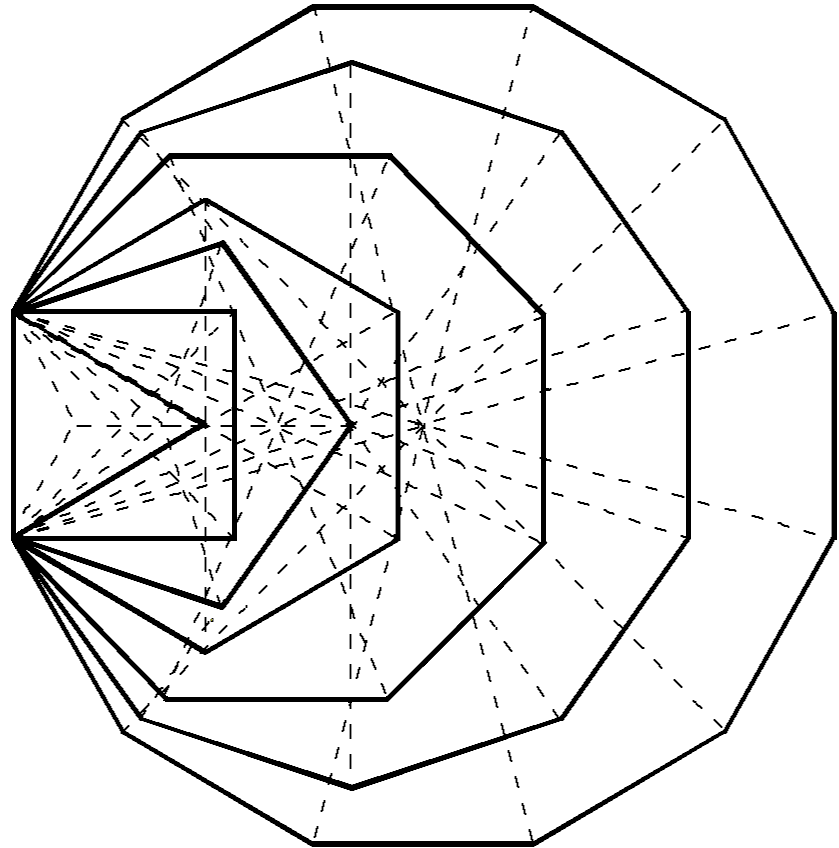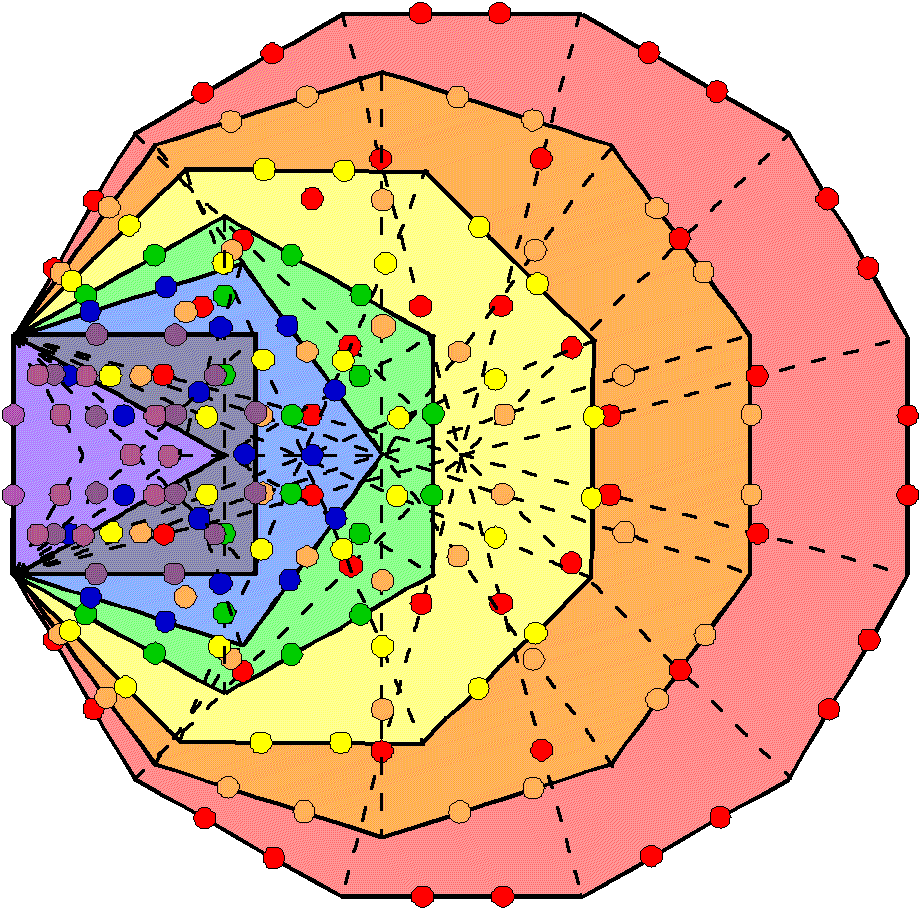The 7 enfolded polygons are composed of 176 corners, sides & triangles. 176 hexagonal yods line the 88 sides of the 47 tetractyses in the 7 enfolded polygons. Figure 7. 236 yods line the boundaries of the (7+7) enfolded polygons. Figure 8. The number 176 is a structural parameter of the inner Tree of Life.

corners of the (7+7) enfolded polygons that belong to the set of 236 boundary yods. The yod population of the 5-cell is the number of yods shaping the inner Tree of Life. Moreover, 176 yods line its 60 tetractyses. This is both the number of geometrical elements in the 47 sectors of the seven enfolded polygons and the number of hexagonal yods on the 88 sides of the 47 tetractyses (Fig. 8). In the former, the sum “1 + 175” appears as the topmost corner of the hexagon that coincides with the lowest corner of the hexagon enfolded in the next higher Tree and as the 175 geometrical elements intrinsic to the seven enfolded polygons. In the latter, the sum appears as one of the two hexagonal yods on the root edge that can be associated with one set of seven polygons and as the 175 hexagonal yods lining their 47 tetractyses. The possession of Tree of Life parameters by the 5-cell is strong evidence of its holistic nature as the simplest polychoron.

2) The 5-cell contains 210 (=21×10) hexagonal yods, where 21 is the number value of EHYEH, the Godname of Kether. It has 15 corners in its 10 faces and 11 corners in its interior, totalling 26, where 15 is the number of YAH and 26 is the number of YAHWEH.

8-cell

1) It has 73 corners of 192 tetractyses, where 73 is the number value of Chokmah.

2) 296 yods line the 96 tetractyses in its 24 faces. 16 of these are vertices, leaving 280 yods needed to create these faces, where 280 is the number value of Sandalphon, the Archangel of Malkuth.

16-cell

1) 280 yods line the tetractyses. This number reappears because the 16-cell has eight fewer vertices than the 8-cell but eight more faces, whilst it has eight fewer edges, so that it has (8×2=16) fewer yods in its faces, i.e., the same number of yods as in the 8-cube other than its 16 vertices.

2) Its 168 tetractyses have 65 corners. This is a remarkable conjunction of the numbers of the Godname and Mundane Chakra of the same Sephirah (Malkuth). 64 (=43) corners surround its centre.

3) Its 32 faces have 336 hexagonal yods. This number has been discussed in many previous articles as a superstring structural parameter, being the number of turns in one revolution of each helical whorl of a UPA around its axis of spin.

24-cell

1) 216 corners of 576 (=242=12×22×32×42) tetractyses surround its centre, where 216 is the number value of Geburah. 120 of them (24 vertices) are in its 96 triangular faces, where 120 is the sum of the first 10 odd integers after 1. The 24-cell consists of 24 octahedral cells.

120-cell

1) It has 1320 (=132×10) yods at corners of 3600 tetractyses in its 720 pentagonal faces. 132 is the 66th even integer, where 66 is the 65th integer after 1 and 65 is the number value of ADONAI, the Godname of Malkuth.

600-cell

1) The number of yods in its 1200 faces is 13560, that is, the number of yods in 1356 tetractyses. The

11

number 1356 is the number of yods surrounding the centres of the 14 enfolded Type B polygons, which contain 1370 yods (see Fig. 4). 13560 yods surround the (70+70=140) centres of the (70+70) polygons enfolded in 10 separate Trees of Life. This is remarkable evidence for the holistic character of the 600-cell, for in each case this number determines the form of the two equivalent geometries. The inner Tree of Life shares with its outer form seven yods on the vertical diameter of each hexagon, one of which is the centre of this polygon, and the centre of each triangle. Each set of seven polygons shares six yods that are not centres of polygons. The number of these shared yods in the (7+7)n polygons enfolded in the n-tree = 5n + 1 + 5n +1 = 10n + 2. The number of yods in these polygons = 1368n + 2. The number of yods surrounding their centres = 1368n + 2 – 7n – 7n = 1354n + 2. The number of such yods that are intrinsic to the polygons in the sense that they are unshared with the n-tree = 1354n + 2 – (10n+2) = 1344n. The (70+70) polygons enfolded in the 10-tree have 13440 intrinsic yods surrounding their 140 centres. This is the number of yods other than vertices in the faces of the 600-cell: 13560 – 120 = 13440. Given the 10-tree and the 140 centres of the polygons making up its inner form, 13440 more yods are needed to construct the latter from Type B triangles. In an analogous way, given the 120 vertices of the 600-cell, 13440 more yods are needed to construct it in 4-dimensional space. Discounting the possibility of coincidence as implausible, this suggests that the 600-cell is the 4-dimensional polychoron counterpart of the inner form of 10 overlapping Trees of Life. The archetypal nature of the latter has been analysed in depth in many previous articles, as has its equivalence to other sacred geometries, such as the disdyakis dodecahedron. Moreover, the analogy is unique, for the faces of the 120-cell (the only other polychoron whose yod population is an integer multiple of 10) have 14520 yods — more than the 13682 yods in the (70+70) polygons enfolded in the 10-tree.

2) The number of yods lining the 720 edges connecting its 120 vertices = 120 + 2×720 = 1560 = 156×10, where 156 is the 155th integer after 1. ADONAI MELEKH, the Godname of Malkuth with number value 155, appropriately prescribes the form of the 600-cell.

3) 21000 (=21×1000) yods surround its centre. This is a remarkable example of how Godnames prescribe objects with holistic character (as we shall see shortly), for 21 is the number value of Kether, the first Sephirah.

4) The 600-cell is composed of 600 tetrahedra, joined five to an edge.

5) 9960 yods line the 3600 tetractyses in its faces. Of these, 1560 yods line the 720 edges. This leaves (9960−1560=8400) more boundary yods needed to construct its 1200 faces from Type A triangles. This is the same as the number of new geometrical elements needed for their construction, as found in the discussion of the 600-cell in Section 2. Once again, the superstring structural parameter 8400 appears as the number of yods lining the 3600 tetractyses other than those on its edges.

4. The 421 polytope
Firstly, we need to consider a few geometrical concepts. A skew polygon, is a polygon whose vertices do not lie in a plane. Skew polygons must have at least four vertices. A regular skew polygon has equal edge lengths. A facet of a polyhedron is any polygon whose corners are vertices of the polyhedron, and need not be a face. More generally, a facet of an n-polytope is an (n–1)-polytope formed by some of its vertices. n-dimensional facets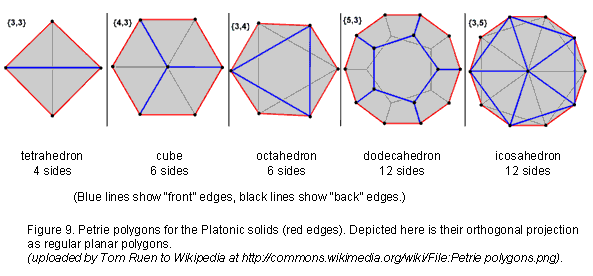are also called n-faces. For example, a 0-dimensional face is a vertex of a polyhedron, a 1-face is a 1-dimensional edge and a 2-face is a 2-dimensional face of a polyhedron. In higher-dimensional geometry, the facets of a n-polytope are the (n-1)-faces of dimension one less than the polytope itself. A Petrie polygon for a regular polytope of n dimensions is a skew polygon such that every (n1) consecutive sides (but no n) belong to one of the facets. For every regular polytope, there exists an orthogonal projection onto a plane such that one Petrie polygon becomes a regular polygon

12

with the remainder of the projection interior to it. The plane in question is the Coxeter plane of the symmetry group of the polygon. Figure 9 shows the Petrie polygons for the five Platonic solids.

Shown in Figure 10 are the Petrie polygons of the six polychorons.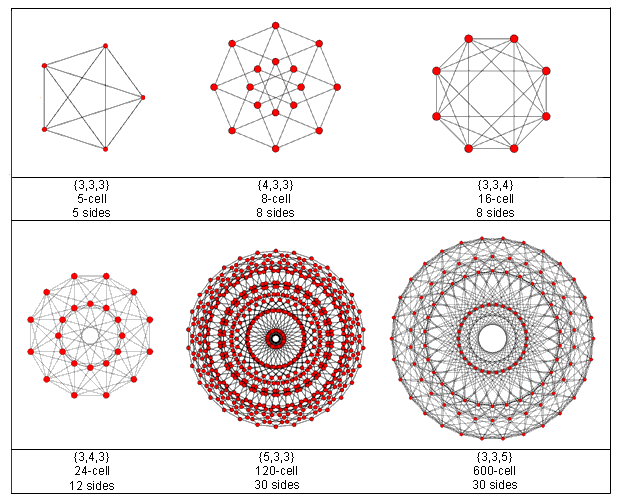Figure 10. The Petrie polygons of the six polychorons.

It is amusing that the pentagram, which the ancient followers of Pythagoras displayed on their arms as a public sign of their membership in his brotherhood, should appear in the Petrie polygon of the 5-cell, which is the simplest, 4-dimensional, regular polyhedron. They would certainly have approved, given their emphasis of the central role of the number 4 in the mathematics that describes nature, as enshrined in the author’s Tetrad Principle formulated in Article 1 and as demonstrated in nearly every one of his previous articles! The Petrie polygon of this polyhedron is a simple pentagon because the 5-cell is dual to itself.

In 1900, Thorold Gossett (1869-1962), an English lawyer and amateur mathematician, discovered  an 8‑dimensional, semi-regular polytope that has proved to be relevant to the theory of superstrings (or at least to the mathematics of one of the two symmetry groups describing superstring forces). The n-face composition of the so-called "421 polytope" is shown below in Table 6:

Table 6. Numbers of n-faces in the 421 polytope.

 Vertices (0-faces) Edges (1-faces) Faces (2-faces) {3} Cells (3-faces) {3,3} 4-faces {3,3,3} 5-faces {3,3,3,3} 6-faces {3,3,3,3,3} 7-faces {3,3,3,3,3,3} + 411 Total 240 6720 60480 241920 483840 483840 207360 17280 + 2160 = 19440 1503840

It has 6720 edges, where 6720 = 672×10. Compare this with the fact that the first four Platonic solids:

tetrahedron, octahedron, cube & icosahedron

13Figure 11. The inner form of 10 Trees of Life has as many yods as that which line the edges of the 421 polytope.

have 672 yods when their faces and interiors are constructed from tetractyses . It is an amazing example of how the same parameters quantifying global properties of holistic systems appear in different sacred geometries. Another example is the following: suppose that we turn each triangular face into a tetractys. The number of yods lining its edges = 240 + 6720×2 = 13680. As was pointed out in the earlier discussion of the properties of the 120-cell (see comment 3), the (7+7) enfolded Type B polygons of the inner Tree of Life have 1368 intrinsic yods, so that the (70+70) Type B polygons enfolded in 10 overlapping Trees of Life have 13680 yods intrinsic to them (Fig. 11). We see that the number measuring the shape of the 421 polytope as the number of yods aligning its edges is exactly the same as the yod population of the inner form of 10 overlapping Trees! Moreover, the (7+7) enfolded polygons have 26 yods that are either centres or yods shared with the outer Tree. Of these, two yods are corners of hexagons that coincide with corners of hexagons enfolded in the next higher Tree, so that 24 of these yods belong to each set of (7+7) polygons. It means that 240 yods in the 140 polygons enfolded in 10 overlapping Trees of Life are either centres or shared. They correspond to the 240 vertices of the 421 polytope. (13680–240=13440) yods intrinsic to the (70+70) polygons surround their centres. They correspond to the 13440 hexagonal yods lining the 6720 edges of the 421. Such correspondences make the conclusion seem inescapable that the 421 polytope central to superstring theory has archetypal character because it is the polytope analogue of the inner form of 10 overlapping Trees of Life, each representing a Sephirah. Figure 12 shows its Petrie projection onto the Coxeter plane. Its Petrie polygon is a 30-gon.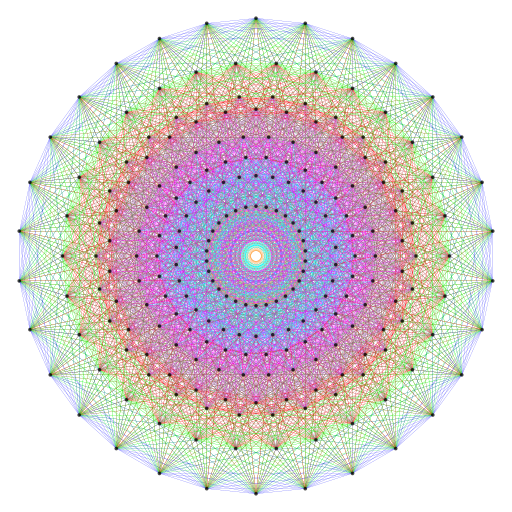Figure 12. Petrie projection of the 421 polytope. (Place cursor over image to enlarge it).

As found earlier, the 1200 faces of the 600-cell contains 9240 points, lines & triangles when constructed from Type A triangles. They comprise the original 840 vertices & edges and 8400 new points, lines & triangles generated from the seven points, lines & triangles inside each face.

14

The 2400 faces of two such polytopes comprise (840+840=1680) vertices & edges and (8400+8400=16800) new points, lines & triangles (4800 points or lines and 3600 triangles, or 4800 points or triangles and 3600 sides). What this means is that 8400 geometrical elements are needed to construct the faces of each 600-cell from simple triangles in a way that is consist with the construction of other polytopes from triangles, a requirement which demands that all polygonal 2-faces be divided into their sectors. They comprise 1×1200 points, 3×1200 lines and 3×1200 triangles, that is, 7×1200 geometrical elements. Many previous articles have shown that this inner form embodies the numbers 840 and 1680, and now we encounter these very numbers in the numbers of vertices & edges making up the two concentric 600-cells whose 240 vertices are root vectors of E8. As these numbers are the numbers of circular turns in, respectively, an outer or inner half of a helical whorl and a whole whorl, the inevitable conclusion to be drawn is that the UPA is, indeed, an E8×E8 heterotic superstring the symmetry of whose forces is described by E8. In other words, the 421 polytope embodies not only the 240 roots of E8 but also the number of circular turns in the 10 helical whorls along which the 240 E8 gauge charges are spread. Each of the 16800 circular turns would correspond to one of the 16800 geometrical elements within the faces of the two 600-cells generated by their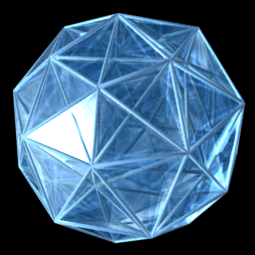Figure 13. Zome model of the 600-cell as a compound of five 24-cells. (Credit: http://homepages.wmich.edu/~drichter/five24cells.htm). 3-dimensional projection of 600-cell. (Credit: "600-cell" by Jason Hise, uploaded to Wikipedia.org.).

construction from triangles. One of the 600-cells with 120 vertices and 840 vertices & edges would correspond to the outer half of the UPA as its 10 whorls make 25 revolutions, the 2½ revolutions in each helical whorl comprising 840 turns, and the other 600-cell with 120 vertices would correspond to its inner half as its 10 whorls make another 25 revolutions. The appearance of the numbers 840 and 1680 in the two 600-cells making up the 421 polytope is sufficient to establish the identity of the UPA as an E8×E8 heterotic superstring. The appearance of the numbers 8400 and 16800 in their construction from Type A triangles is, so, to speak, the “icing on the cake.” They refer to both the geometrical composition and boundary yods of the two 600-cells, for — as we found earlier — 8400 yods lining tetractyses in addition to those on edges are needed to construct the faces of each 600-cell, so that (8400+8400=16800) extra boundary yods are needed to create the faces of both 600-cells making up the 421 polytope.

It is known to mathematicians that the 120 vertices of a 600-cell can be portioned into those of five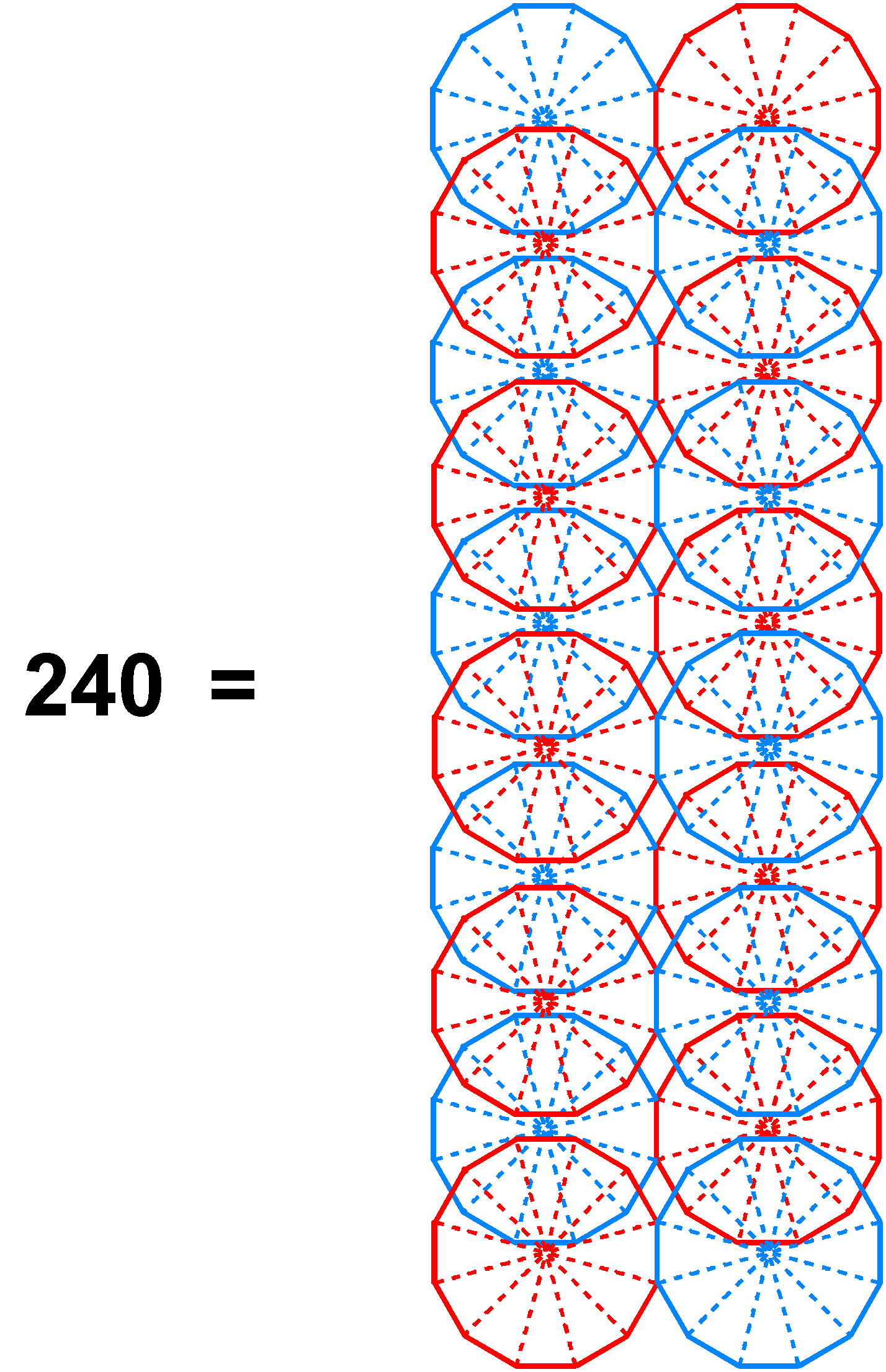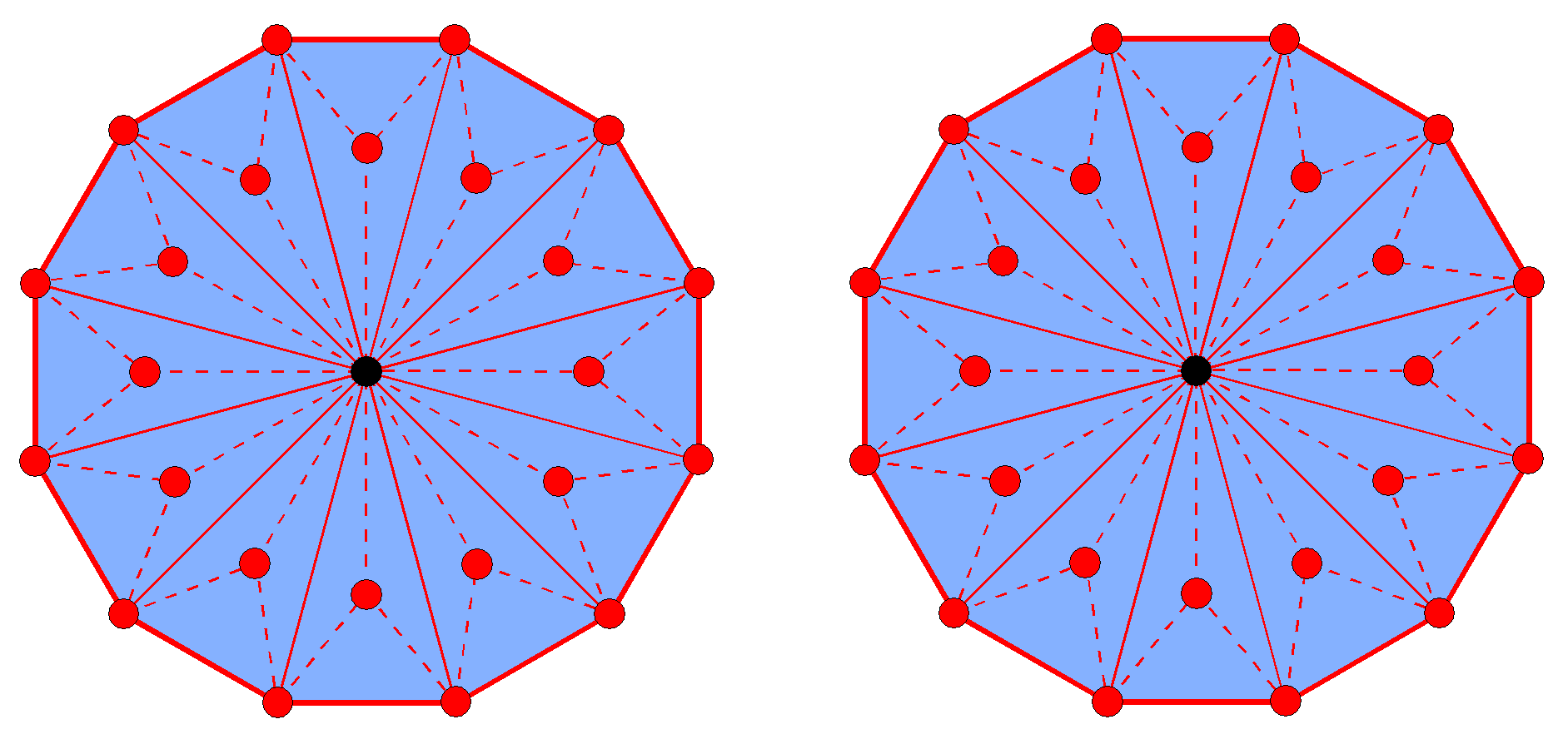Figure 15. (120+120=240) points, lines & triangles surround the centres of the two separate Type B dodecagons.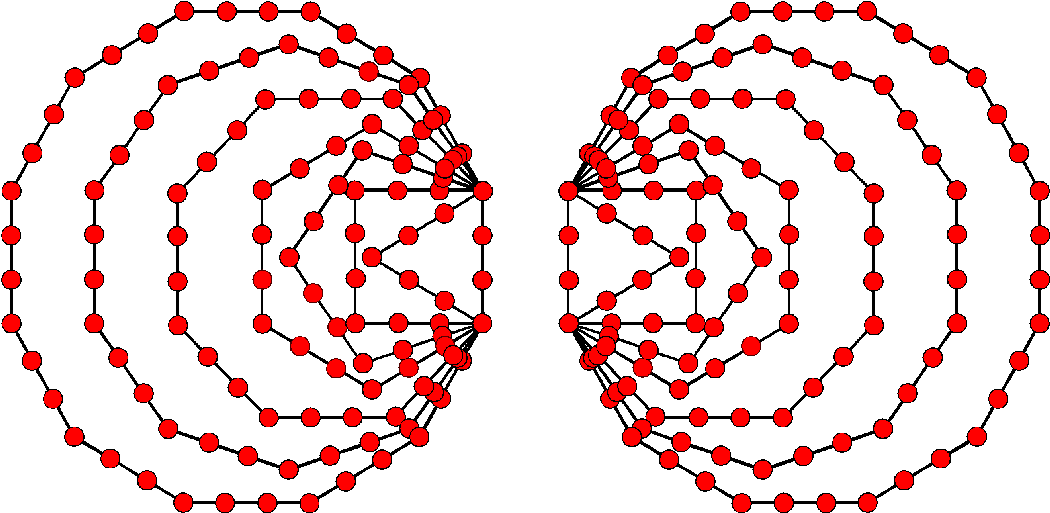Figure 14. The (10+10) Type A dodecagons enfolded in 10 Trees of Life have 240 sectors with 240 corners. 120 corners are associated with each set of 10 dodecagons. Figure 16. (120+120=240) yods line the sides of the (7+7) polygons enfolded in the separate halves of the inner Tree of Life.

15

disjoint 24-cells, each with 24 vertices (Fig. 13). This compares with the fact that the dodecahedron with 20 vertices is formed from five distinct tetrahedra. The two 600-cells comprise 10 disjoint 24-cells. This 10-fold character was shown in Article 53 to be a natural property of sacred geometries, indicating that it is the geometrical basis of either the 10 whorls of the UPA or its 10 half-revolutions. A similar property now emerges in the geometry of the 421 polytope whose 4-dimensional projection is the compound of two 600-cells. As we saw earlier, it has its striking counterpart in the inner form of 10 overlapping Trees of Life as the 10 sets of 24 yods that are either centres of the (70+70) enfolded polygons or shared with these Trees. This 10-fold pattern in the E8 lattice of 240 roots suggests that the whorl “carries” 24 E8 gauge charges corresponding to 24 roots that are represented by the 24 vertices of a 24-cell. The UPA would have 10 whorls because the two 600-cells consist of 10 disjoint 24-cells. Alternatively, a 24-cell could represent a half-revolution of all 10 whorls, the two 600-cells determining the outer and inner halves of the UPA, each of which comprises 2½ revolutions of 10 whorls around the axis of the UPA, the five half-revolutions being represented by the five 24-cells.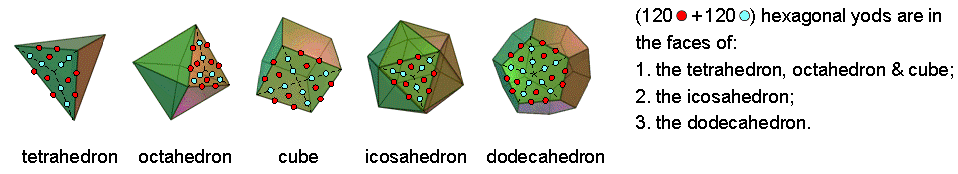Figure 17. The 120:120 pattern in the faces of the five Platonic solids.

That the physics of matter might be determined by the (120+120=240) vertices of two concentric 600-cells that are the projection of the 240 root vectors of the lattice of E8 is indicated by the two dodecagons in the inner form of the Tree of Life. When they are Type A, their 24 sectors have 24 corners, so that the (10+10) dodecagons enfolded in 10 Trees of Life have 240 sectors with 240 corners (Fig. 14). 120 corners can be associated with either set. Moreover, the centre of a Type B dodecagon is surround by 120 points, lines & triangles, 10 geometrical per sector, so that the two dodecagons belonging to the inner Tree of Life comprise (120+120=240) geometrical elements arranged in 10 sets of 24 elements (Fig. 15). In fact, 120 yods line the sides of each set of seven enfolded polygons (Fig. 16), whilst the first four Platonic solids have 480 hexagonal yods in their faces, the tetrahedron, octahedron & cube having 240 hexagonal yods and the icosahedron having 240 hexagonal yods (Fig. 17). Both the icosahedron and the dodecahedron have 120 hexagonal yods in the faces of each half of the polyhedron, as do the first three solids. It is easily verified that each set of 120 hexagonal yods comprise 10 sets of 12, so that the 240 hexagonal yods in the faces of the first three Platonic solids comprise 10 sets of (12+12=24) hexagonal yods, as do the last two solids (for more examples, see Article 53).

Many previous articles have shown that the Catalan solid called the “disdyakis triacontahedron” is the polyhedral counterpart of 10 overlapping Trees of Life. It has 62 vertices (30 A vertices, 12 B vertices & 20 C vertices) and 180 edges that are of three types. Sixty edges join A & B vertices, 60 edges join B & C vertices and 60 edges join C & A vertices. The B vertices are vertices of an icosahedron and the C vertices form a dodecahedron. Surrounding an axis that passes through two diametrically opposite B vertices are 20 C vertices, 30 A vertices & 10 B vertices, i.e., 10 sets of six vertices (3A, B & 2C).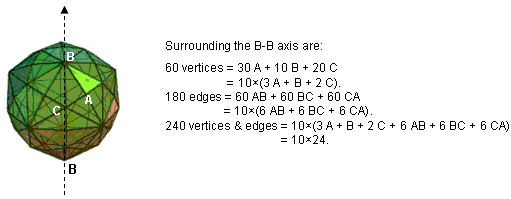Figure 18. Surrounding an axis of the disdyakis triacontahedron are 10 sets of 24 vertices & edges.

Surrounding the axis are 10 sets of six AB edges, 10 sets of six BC edges & 10 sets of six CA edges.

16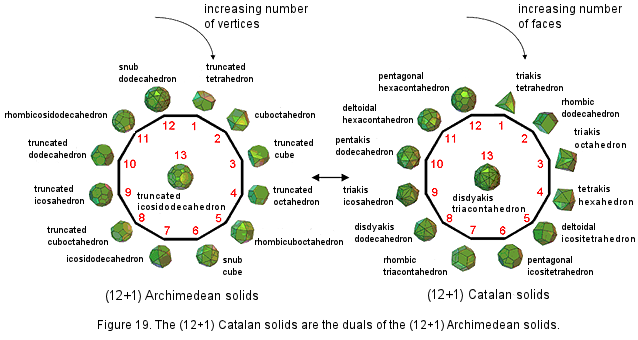Therefore, the axis is surrounded by 240 vertices & edges comprising 10 sets of 24 vertices & edges, each set comprising six vertices (3A, B & 2C) and 18 edges (6AB, 6BC & 6CA) (Fig. 18). The disdyakis triacontahedron therefore manifests the same 10×24 pattern as the Platonic solids, the inner Tree of Life and the 421 polytope. In fact, the pair of separate dodecagons not only generates the 10×24 andFigure 20. The connection between the UPA/superstring, the 421 polytope and 4-dimensional polytopes.

17

(120+120) patterns characteristic of sacred geometries through their (12+12) sectors but also the 13 Archimedean solids and their duals (the Catalan solids) can be assigned to the 26 corners of these sectors, the disdyakis triacontahedron and its dual (the truncated icosidodecahedron) being assigned to the centres of the two dodecagons (Fig. 19).

The compound of two 600-cells that is the 4-d projection of a 421 polytope  is the 4-dimensional manifestation of this archetypal pattern exhibited by sacred geometries. Constructed from Type A triangles, it contains 4080 points, where

4080 = (24 + 25 + 26 + 27) + (28 + 29 + 210 + 211),

240 = 24 + 25 + 26 + 27

is the number of vertices of the two 600-cells and

3840 = 28 + 29 + 210 + 211

is the number of other corners of their triangles. The two 600-cells have 13200 sides of 11520 triangles, that is, 11760 sides other than their 1440 edges, where 11760 = 1680×7. This is the number of turns in the seven helical minor whorls of the UPA. Each cell has 5760 triangles with 6600 sides, where 6600 = 55×120. The number 55 is the sum of the first 10 integers and 120 is the sum of the first 10 odd integers after 1, showing how the Decad determines the number of straight lines constructing the 600-cell.

This pattern manifests in the subatomic world in the E8×E8 heterotic superstring as the 120 E8 gauge charges that are spread along the inner and the outer halves of the 10 whorls of the UPA, 12 charges per half-whorl (Fig. 20). The composition of each 600-cell representing the inner or outer half of the UPA in terms of five 24-cells determines the five half-revolutions of each half. As 16800 = 240×70, it means that each E8 gauge charge manifests as 70 turns of a helical whorl. This is both the number of yods in the Tree of Life created from tetractyses and the number of corners of the (7+7) enfolded polygons of its inner form. As might be expected, each charge is a whole in itself and so must be represented by a Tree of Life whose 16 tetractyses are composed of 70 yods. The boundary of the 24-cell is composed of 24 octahedral cells with six meeting at each vertex, and three at each edge. Together they have 96 triangular faces, 96 edges, and 24 vertices. The vertex-first parallel projection of the 24-cell into 3-dimensional space has a rhombic dodecahedral envelope. Twelve of the 24 octahedral cells project in pairs onto six square dipyramids that meet at the centre of the rhombic dodecahedron. The remaining 12 octahedral cells project onto the 12 rhombic faces of the rhombic dodecahedron.

The superstring structural parameter 1680 and its connection to the 240 roots/gauge charges of E8 manifests in another context. The sphere in 3-dimensional space R3 has a 2-dimensional surface S2. In (n+1)-dimensional space Rn+1, the n-sphere Sn with radius r is the set of points {x1,x2,…xn+1} equidistant from a point {c1,c2,…cn+1} such that

 n+1 r2 = ∑ (xi − ci)2 i=1

A question that has occupied mathematicians for many years is: how many n-spheres can be packed in Rn+1 so as to touch a central n-sphere? This is called the “kissing number problem,” the number K(n) being called the “kissing number.” In 1-dimensional space, the 0-sphere is a point and the answer is 2 because a point at the middle of a finite straight line is equidistant from the two end points of this line. In 2-dimensional space, the 1-sphere is a circle and six similar circles can be arranged with their centres at the corners of a hexagon so as to touch a circle of the same radius at its centre. In 3-dimensional space, K(3) = 12, although it took until 1953 to prove this rigorously. In 4-dimensional space, K(4) = 24 (first proved in 2003 by Oleg Musin ). The densest lattice packing of spheres in this space is for 24 3-spheres centred on the vertices of a 24-cell. It explains why the 600-cell with 120 vertices can be regarded as five disjoint 24-cells. The problem is still unsolved for general n>4, although various upper and lower limits for K(n) have been derived for certain value of n. However, the kissing number problem is exactly solvable for spheres in eight and 24 dimensions. It was first proved in 1979 that K(8) = 240. Then a Ukrainian mathematician, Maryna Viazovska, proved in March, 2016 that the packing of 240 spheres in the 8-dimensional E8 lattice space is the densest possible . Amazingly, the number of 7-spheres that can touch a central sphere in 8-dimensional space is the same as the number of vectors of the 8-dimensional root lattice space of E8! Not only that, their centres coincide with its 240 lattice points! A point in 8-dimensional space has the spherical coordinates (r, φ1, φ2, φ3, φ4, φ5, φ6, φ7). The positions of points on 240 7-spheres require (240×7=1680) angular coordinates to specify them. This is the number of 1st-order spirillae in a whorl of the UPA that Leadbeater painstakingly counted 135 times . It is another way in which this superstring structural parameter appears naturally in the group mathematics of E8×E8 heterotic superstrings.

Of course, the author does not claim that this property is the complete explanation of the string winding number 1680. This because the number refers to a property of spheres packed in a special,

18

8-dimensional lattice, whereas in the context of the UPA it refers to the number of times a closed curve winds around the axis of a 2-torus. The crucial point is that it is highly unlikely that it could be just by chance that this number with an alleged paranormal provenance appears naturally twice in a narrow context connected to the group mathematics of superstrings, namely, the 421 polytope, whose vertices determine the 240 roots of E8 whose 4-d projection is the compound of two 600-cells with 1680 vertices & edges, and the specification of the 240 root vectors of E8 by 1680 angular coordinates. The argument against coincidence has even more force vis-à-vis the UPA's structural parameters 840, 8400 & 16800, which we have seen manifest uniquely in this polytope. The conclusion is inescapable that the UPA is a state of the E8×E8 heterotic superstring and that both its space-time structure and the symmetry of its forces are laid out in the 4-dimensional sacred geometries discussed in this article, as well as in the 3-dimensional sacred geometries discussed in previous articles.

5. The Tetrad determines the superstring structural parameter 16800

According to Table 3, 14400 geometrical elements surround the centre of a 600-cell constructed from Type A triangles. Two 600-cells contain 28800 geometrical elements surrounding their centres, where 28800 = 1!×2!×3!×4!(13+23+33+43). According to Table 5, the centre of a 600-cell is surrounded by 21000 yods, so that the centres of two 600-cells are surrounded by 42000 yods, where 42000 = 1680×25. Noting that 168= 132− 1 = 3 + 5 + 7 +... + 25, i.e., it is the sum of the first 12 odd integers after 1, the superstring structural parameter 1680 is the sum of the 12 integers 30, 50, 70, ... 250 that can be assigned to the 12 yods on the boundary of a Type A square, which has 25 yods, whilst 4200 is the sum of the same integer 1680 assigned to all its yods:If the sceptic thinks that it is just coincidence that the number of vertices & edges in two 600-cells should be the structural parameter of the basic particle that C.W. Leadbeater claimed to remote-view, despite their being connected to the 421 polytope representation of the roots of the symmetry group E8 in E8×E8 heterotic superstring theory, then — to remain consistent — he has to accept that it is also just a matter of chance that the square should simultaneously generate this number and the yod population of the two 600-cells. How many such miraculous coincidences is he willing to accept before he grudgingly admits that a profound, mathematical design embodied in the UPA is being revealed here? Any argument based upon chance that he uses to justify his scepticism is untenable, given the degree of correlation established in this article between the features of the UPA, the geometry of the two 600-cells as the 4-dimensional projection of the 421 polytope and the five-fold distribution of their vertices in 24-cells.

The Pythagorean integers 1, 2, 3 & 4 determine the 2400 faces of the two 600-cells because

 11 12 13 14 43 44 21  21 22  22 23  23 24  24 33  33 34  34 2400 = 31  31  31 + 32  32  32 + 33  33  33 + 34  34  34 + 23  23  23 + 24  24  24 41  41  41  41 42  42  42  42 43  43  43  43 44  44  44  44 13  13  13  13 14  14  14  14

They determine the number 1680 because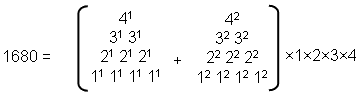They determine the number 16800 because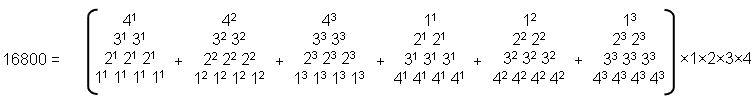19

6. The holistic 72:168 division in the two 600-cells making up the 421 polytope
As additional evidence of the holistic nature of the compound of two 600-cells, it will be next proved that the yod composition of their faces displays the 72:168 division characteristic of holistic systems.

Table 5 indicates that the 1200 faces of the 600-cell contains 13560 yods. Its 720 edges comprise (120 + 2×720 = 1560) yods. (13560−1560=12000) new yods are needed to turn the faces into Type A triangles. Of these, 3600 hexagonal yods are at the centres of the (3×1200=3600) tetractyses, leaving 8400 new yods on their sides. Therefore, 12000 = 3600 + 8400. (2×12000=24000) new yods are needed to construct the 2400 faces of the two 600-cells. They contain (2×3600=7200) hexagonal yods at the centres of the 7200 tetractyses and (2×8400=16800) new yods on their sides. Hence:

24000 = 7200 + 16800.

Ignoring the Pythagorean double factor of 100 originating from the fact that the two 600-cells have 2400 (=24×100) faces, we see that the compound of two 600-cells exhibits the 72:168 division that is found in all other sacred geometries. Moreover, the 36:84 division differentiating between central hexagonal yods and boundary yods is exactly the same as the division of the 120 yods on the boundaries of each separate set of seven enfolded polygons into 36 corners and 84 hexagonal yods (Fig. 21).

Article 62 discusses how other sacred geometries manifest this pattern in the holistic parameter 240. The fact that it is revealed in the very compound of two 600-cells that generates the 421 polytope when they are constructed from tetractyses is truly remarkable evidence of the archetypal nature of this polytope as sacred geometry. In terms of the 240 roots of E8, this division is between the 72 roots of its exceptional subgroup E6 and its 168 remaining roots. Here is the sacred geometrical counterpart of this property.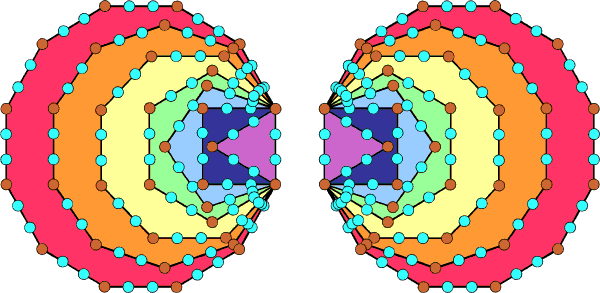Figure 21. The 120 yods lining the sides of each set of 7 enfolded polygons consist of 36 brown yods at their corners and 84 turquoise hexagonal yods.

7. The holistic 192:192 division in the two 600-cells

We found in comment (2) on the 600-cell that the centre of a 600-cell is surrounded by 1920 corners of sectors in its 1200 faces and 720 interior triangles that are not vertices. Therefore, (192+192=384)×10 points other than vertices surround the common centre of two 600-cells. As

 12 22 32 385 = 42 52 62 72 82 92 102,

384 is the sum of the squares of 2-10, so that 1920 = 5×(22 + 32 + ... + 102). This means that the sum of the 90 squares of 2-10 arranged in a 10-fold array surrounding the central square of 1 (Fig. 22) is 3840 — the number of corners other than vertices of the 7200 sectors of the 2400 faces of two 600-cells. This demonstrates the remarkable way in which the Decad determines the number of points other than vertices that surround the common centre of the two 600-cells.

Alternatively, if the 120 vertices of the 600-cell are joined to its centre, creating the sides of triangles, and the internal triangles and the faces treated as simple triangles, then there are (1200+720=1920) triangles and 1920 lines & triangles in its faces. It has (120+720=840) internal sides & triangles, as well

20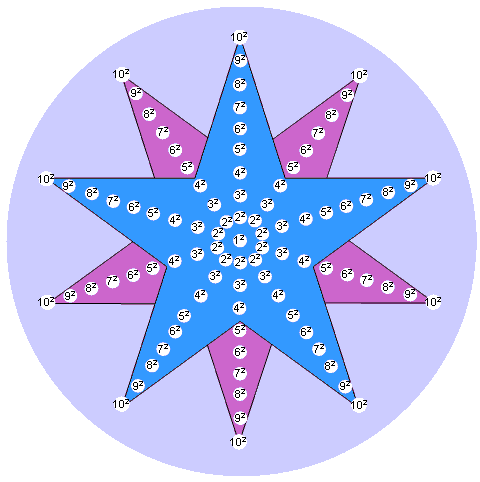Figure 22. Each pentagram array of the squares 22-102 sums to 1920. This is the number of corners of triangles in each 600-cell that are not its vertices or, alternatively, the number of its external and internal triangles when both types are simple triangles.

as (720+120=840) external & internal lines and (120+720=840) points & lines in its faces. Therefore, there are three possible sets of 840 geometrical elements: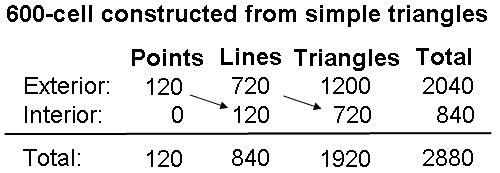Assuming that their sets of 840 elements are compounded in the same way, the two 600-cells sharing the same centre are composed of four possible sets of 1680 geometrical elements:

1. (840+840) points & lines in their 2400 faces;
2. (840+840) external & internal lines;
3. (840+840) internal lines & triangles;
4. (840+840) points & triangles.

There can, therefore, be no doubt whatsoever that the pair of 600-cells embodies the paranormally obtained superstring structural parameter 1680. It does not need its construction from Type A triangles to demonstrate that. But the latter is required to show that the compound embodies the numbers 8400 and 16800 as well. Notice that even a single 600-cell can embody the number 1680, for its faces are composed of 840 points & lines, whilst its interior has 840 lines & triangles. Alternatively, it has 840 lines and 840 points & internal triangles. The question now arises: which of all these possibilities is the correct geometrical analogue of the 1680 turns in a helical whorl of the UPA? To answer this question, it is necessary to point out that it is not sufficient that a parameter of holistic systems be embodied in a geometry for the latter to count as sacred. True parameters of holistic systems are not isolated; they are also interconnected, forming patterns that are always the same from one holistic system to another. Study of these systems has shown that one such pattern is the sum:

192 = 24 + 168

21

(or a pattern with 10 times these numbers). A holistic parameter may be present in a sacred geometry in more than one way. Its "correct" way will be that which is consistent with the holistic patterns that the geometry displays. In the case of the 600-cell constructed from simple triangles, there are (720+1200=1920) lines & triangles in its faces and (720+1200=1920) triangles in its faces and interior. In neither case, however, does this number include the number 240. This appears only as the sum of the 120 vertices and the 120 internal lines, but in each case the number 120 is also part of the number 840 instead of being separate from it, which it needs to be in order to create the sum: 1920 = 240 + 1680. This holistic pattern only manifests in the pair of 600-cells, which contains 240 points (vertices) and 1680 lines (edges & internal lines), i.e., 1920 points & lines. As we have found that sacred geometries embody the numbers 384 and 192 (or 10 times these numbers) as:

384 = 192 + 192,

it indicates that the truly complete, holistic system exhibiting the division: 3840 = 1920 + 1920 is one in which the compound is replicated, i.e., there should be two 421 polytopes, each with 240 vertices determining the 240 roots of E8. But this is, precisely, the E8×E8 heterotic superstring theory, which this website demonstrates is encoded in the inner Tree of Life, the five Platonic solids and the disdyakis triacontahedron! Hence, this particular superstring theory is the simple consequence of holistic systems having two suitably defined "halves", each embodying the number 192 (or 1920). The correct way of seeing how the superstring structural parameter 1680 is embodied in either the 600-cell or two 600-cells composed of simple triangles is the one that is consistent with both the sums: 1920 = 240 + 1680 and 1680 = 840 + 840. The only one is a compound of two 600-cells with 240 points, 1680 lines and 1920 points & lines, i.e., each 600-cell with 840 sides of external and internal simple triangles represents half a helical whorl with 840 turns. This is consistent with the conclusion reached in Article 62 that, in terms of its vertices, the two 600-cells composed of Type A triangles represent the outer and inner halves of a UPA/E8×E8 heterotic superstring, the 120 vertices in each denoting 120 E8 roots/gauge charges that are spread along either half, whilst each of the five half-revolutions of the 10 whorls in each half is represented by one of the five disjoint 24-cells that make up a 600-cell. The division: 840 = 120 +720 observed for the sides of exterior and interior triangles in each 600-cell is the exact counterpart of what is found in the inner & outer Trees of Life, where 120 yods making up half of the 1-tree constructed from Type A triangles can be associated with the 720 yods that surround the centres of each set of seven separate Type B polygons (see Fig. 5). That the superstring divides into an inner and an outer half due to this 120:120 division of vertices/E8 gauge charges is confirmed by the fact that, when the faces and interior triangles of each 600-cell are Type A triangles instead of simple triangles, (7×1200=8400) geometrical elements are added in the 1200 faces of each 600-cell. In other words, these elements are the counterpart of the 8400 helical turns in the outer or inner halves of the UPA.

8. The Decad determines 120, 1200, 720 & 840

The ancient Pythagoreans regarded 2 as the first true integer because they taught that the number 1 (the Monad) was not a number but the source of all numbers, including integers. They would have treated the number 11, the tenth integer after 1, as the tenth true integer and 3 as the first true odd integer. As

112 − 1 = 121 − 1 = 120 = 3 + 5 + 7 +... + 21,

the Decad determines the 120 vertices of the 600-cell arithmetically as the sum of the first 10 odd integers. It also determines this number in a geometrical way because the centre of a Type B dodecagon is surrounded by 120 points, lines & triangles and the dodecagon is the tenth type of regular polygon. The Decad determines its 1200 faces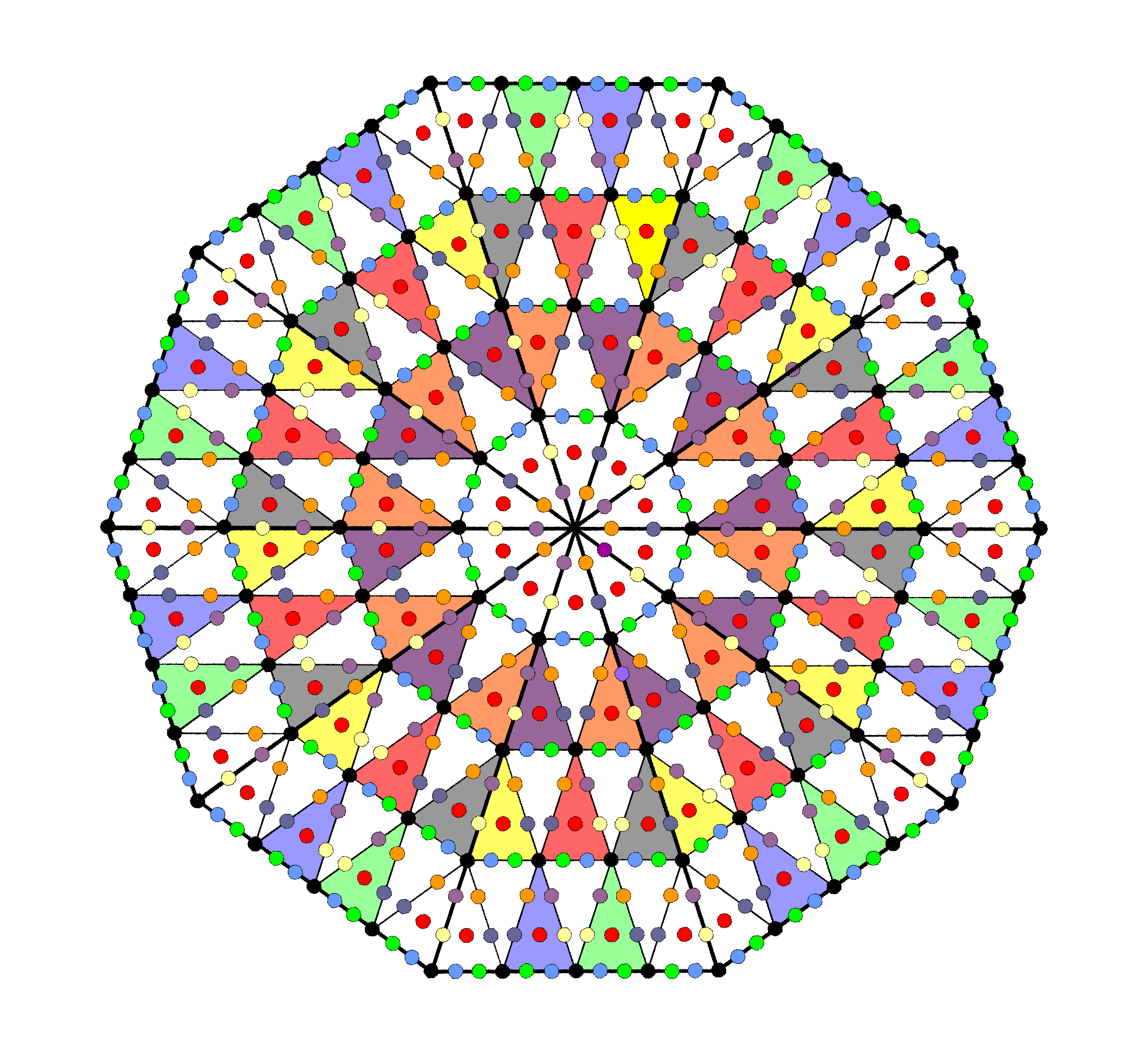Figure 23. 720 yods surround the centre of a decagon whose sectors are 2nd-order tetractyses.

22

because

1200 = 10×120 = 10(3+5+7+... +21) = 30 + 50 + 70 +...+210.

The tenth prime number is 29. As

292 − 1 = 840 = 3 + 5 + 7 +... + 57,

the number 840 is the sum of the first 28 odd integers after 1. Therefore,

840 − 120 = 720 = 23 + 25 + 27 +... + 57,

i.e., 720 is the sum of 18 odd integers. The Decad determines arithmetically both the number of vertices in the 600-cell and its 840 vertices & edges. It determines the 720 edges as well because there are 720 yods surrounding the centre of a decagon whose sectors are 2nd-order tetractyses (Fig. 23).

9. The holistic 168:168 division in the two 600-cells

When the 600-cell is composed of simple tetractyses, (720×2=1440) hexagonal yods line edges of the 1200 tetractyses in the faces and (120×2=240) hexagonal yods line sides of the 720 internal tetractyses. Therefore, (1440+240=1680) hexagonal yods line the 840 sides of the (1200+720=1920) tetractyses. The superstring structural parameter 1680 recorded by C.W. Leadbeater when he remote-viewed the UPA is the number of hexagonal yods in a single 600-cell that correspond to the six Sephiroth of Construction above Malkuth. Here is yet more confirmation of the superstring nature of the UPA. The pair of 600-cells has (1680+1680=3360) hexagonal yods on the 1680 sides of their (1920+1920=3840) tetractyses. This is the number of turns in one revolution of the 10 whorls. Once again, we see that each 600-cell is representing all 10 whorls, but this time a half-revolution of them instead of five half-revolutions. Previous articles have discussed numerous examples of how sacred geometries display the 168:168 or 1680:1680 divisions of their components. The remarkable ways in which polygonal numbers and sacred geometries represent the superstring structural parameter 3360 (number of turns in one revolution of the 10 whorls of the UPA) are shown here.

10. The holistic 120:720 division in two 600-cells and in sacred geometries

Figure 5 displays how the number 1680 is embodied in 1. the disdyakis triacontahedron, 2. the 1-tree & the two sets of 7 separate Type B polygons, and 3. the first four Platonic solids. Their geometrical or yod compositions conform to the pattern:

1680 = 720 + 240 + 720.

This pattern manifests in a single 600-cell composed of simple triangles as the 720 edges, (120+120=240) vertices & internal sides and 720 internal triangles. It appears in two 600-cells as their 240 vertices and their pair of 720 edges. Let us examine these sacred geometries in more detail in order to demonstrate how the holistic 120:720 division exhibited by the 600-cell in both its faces and its interior and by two 600-cells in their vertices & edges manifests in each half of these sacred geometries.

Disdyakis triacontahedron

The polyhedron has 62 vertices, 180 edges & 120 triangular faces. Surrounding an axis passing through two diametrically opposite vertices are 360 (=36×10) geometrical elements comprising 60 vertices, 180 edges, i.e., 240 vertices & edges, and 120 triangles. Joining its vertices to its centre creates internal triangles which, when Type A, are composed of 180 points, (60 + 3×180 = 600) lines & (3×180=540) triangles surrounding the axis. Hence, (60+180=240) points, (180+600=780) lines & (120+540=660) triangles surround the axis. Each half of the disdyakis triacontahedron is composed of 120 points, 390 lines & 330 triangles. i.e., 120 points and 720 lines & triangles. Therefore, the 1680 geometrical elements surrounding the axis consist of two sets of elements, each comprising 840 elements made up of 120 points and 720 lines & triangles. Each half of the disdyakis triacontahedron is the 3-dimensional analogue of a 600-cell.

Outer & inner form of 1-tree
Constructed from 19 Type A triangles, the 1-tree contains 251 yods. Of these, 11 are corners of triangles that coincide with Sephiroth, leaving 240 yods generated by this transformation. The seven regular polygons making up each half of its inner form contain 727 yods when they are Type B. 720 yods surround their centres. It was shown here that the 240 yods in the 1-tree consist of 10 sets of 24 yods. Yods in triangles that are on the left or the right of the central Pillar of Equilibrium can be associated with the yods in the seven regular polygons on that side. The yods that line this pillar as the axis of symmetry of the 1-tree can be divided into two sets containing the same number. Of course, unlike the yods in each set of polygons, these central yods are not mirror images of each other. However, one set can be associated with the left-hand set of polygons and the other set associated with

23

the right-hand set. This means that 120 yods in the 1-tree can be associated with each set of polygons. The 1680 yods can be divided into two sets of (120+720) yods.

First four Platonic solids

How the tetrahedron, octahedron, cube & icosahedron embody the number 1680 is calculated here. When their faces and interiors are constructed from Type A triangles, the numbers of corners, sides & triangles that surround their axes passing through two opposite vertices are tabulated below in Table 7.

Table 7. Geometrical elements surrounding the axes of the Platonic solids.

 Platonic solid Corners Sides Triangles Total Tetrahedron 24 78 66 168 Octahedron 48 156 132 336 Cube 48 156 132 336 Icosahedron 120 390 330 840 Subtotal = 240 780 660 1680 Dodecahedron 120 390 330 840 Total = 360 1170 990 2520

The 1680 geometrical elements surrounding the axes of the first four Platonic solids comprise 240 corners and 1440 sides & triangles. Surrounding the axes of the first three Platonic solids are 120 corners and 720 sides & triangles, i.e., 840 geometrical elements. Similarly, 120 corners and 720 sides & triangles, i.e., 840 geometrical elements, surround the axis of the icosahedron. Amazingly, the first four regular 3-polytopes display the same 120:720 division as the 600-cell, which is the 4-dimensional counterpart of the icosahedron, the fourth Platonic solid! The compound of two 600-cells is the four-dimensional counterpart of the first four Platonic solids. Together with the disdyakis triacontahedron, they are the 3-dimensional and 4-dimensional blueprints for the E8×E8 heterotic superstring, which was paranormally described by Besant & Leadbeater over a century ago. The same pattern is displayed by the icosahedron and the dodecahedron because the latter is the dual of the former and has the same geometrical composition. This is not repeated in their 4-dimensional counterparts because the 120-cell (the 4-dimensional counterpart of the dodecahedron) has 600 vertices, not 120 vertices. It is, however, repeated for its 120 dodecahedra and 720 pentagonal faces. Just as the disdyakis triacontahedron is based upon the rhombic triacontahedron as a compound of the icosahedron and dodecahedron, so the 421 polytope is based upon the compound of two 600-cells. In this sense, it can be regarded as the 8-polytope analogue of the disdyakis triacontahedron — a 3-polytope. What is crucial to recognize is that the highly mathematical, hyper-dimensional objects that are being discovered to underpin E8×E8 heterotic superstring physics have their exact parallels in the sacred geometries of mystical traditions and ancient philosophies. Why? Because they represent the same thing.

11. How the Godnames prescribe the 600-cell and the pair of two 600-cells

Kether: EHIEH = 21.

The first six rows in Pascal's triangle that contain binary coefficients other than 1 comprise 21 such numbers that add up to 240. This is the number of vertices in the two 600-cells: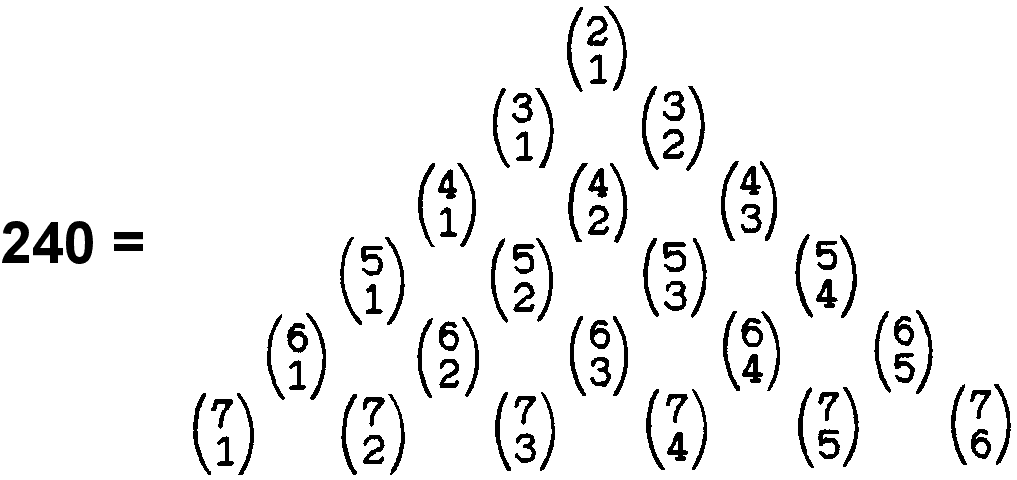The centre of the 600-cell constructed from Type A triangles is surrounded by 21000 (=21×10×10×10) yods.

Chokmah: YAH = 15.

The sum of the first 15 positive integers is 120, i.e., it is the 15th triangular number. This is the number of vertices in the 600-cell. The sum of the first 15 even integers is 240, which is the number of vertices in the two 600-cells. The two 600-cells have 1680 vertices & edges. The sum of the 15 combinations of the four integers 21, 42, 63 & 84 (21 units apart) = 1680. The 15th prime number is 41, where 412 − 1 = 1680.

24

Binah: ELOHIM = 50.

2040 corners of 5760 triangles surround the centre of the 600-cell. Each half has 2880 triangles with 1020 (=102×10) corners. 102 is the 50th even integer after 2.

Chesed: EL = 31.

The two 600-cells have 1680 vertices & edges. 1680 is the sum of the 31 combinations of the five integers 7, 14, 21, 28 & 35, which are 7 units apart. Construction of the faces of the 600-cell from Type A triangles adds (7×1200=8400) geometrical elements. The number 8400 is the arithmetic mean of the 31 square numbers 52, 102, 152  155.2

Geburah: ELOHA = 36.

The 1200 faces of the 600-cell have 3600 (=36×10×10) sectors. It has 720 edges, where 720 = 72×10 and 72 is the 36th even integer.

Tiphareth: YAHWEH ELOHIM = 76.

The pair of 600-cells composed of simple triangles has (1200+1200=2400) triangles in its faces and (720+720=1440) internal triangles, making a total of 3840 (=384×10) triangles. 384 is the 383rd integer after 1, where 383 is the 76th prime number.

Netzach: YAHWEH SABAOTH = 129.
Construction of the 2400 faces of two 600-cells from Type B triangles adds 16800 geometrical elements. As 168 = 132 − 1,

16800 = 168×102 = (132 − 1)102 = 1302 −102 = (2×65)2 − (2×5)2

= 4(652 − 52).

652 = 1 + 3 + 5 + 7 + 9... + 129

and

52 = 1 + 3 + 5 + 7 + 9.

Therefore,

16800 = 4(11 + 13 + 15 +... + 129).

The number 16800 is the sum of (4×60=240) odd integers, the smallest of which is the 10th integer after 1 and the largest of which is 129, the 65th odd integer, where 65 is the sum of the first 10 integers after 1.

Hod: ELOHIM SABAOTH = 153.

1560 (=156×10) yods line the 720 edges of the 600-cell when constructed from tetractyses or Type A triangles. 156 is the 153rd integer after 3, the first odd integer.

Yesod: EL ChAI = 49.

Inside the 600-cell are 2160 triangles with 2280 (=228×10) sides, where 228 is the 227th integer after 1 and 227 is the 49th prime number. Two 600-cells have 2400 faces. As 492 = 2401, 2400 = 492 − 1. This is the dimension of SU(49).

Malkuth: ADONAI = 65.

The 600-cell has (120+720=840) vertices & edges. As 120 = 5! and 720 = 6!, 840 = 6! + 5!. These integers make up the gematria number value of ADONAI. The 3600 triangles in the 1200 faces of the 600-cell have 1320 (=132×10) corners, where 132 is the 65th even integer after 2. The 5760 triangles in its faces and interior have 6600 (=66×10×10) sides, where 66 is the 65th integer after 1.

ADONAI MALEKH = 155.

The number of yods lining the 720 edges connecting the 120 vertices of the 600-cell = 120 + 720×2 = 1560 (=156×10). 156 is the 155th integer after 1.

The sum of the Godname numbers of the first six Sephiroth of Construction is 240, which is the number of vertices of the 421 polytope denoting roots of E8, as well as the number of vertices of the two 600-cells:

240 = 21 + 26 + 50 + 31 + 36 + 76.

The 240 vertices of the 421 polytope can be constructed in two sets: 112 (22×8C2) with coordinates obtained from (±2, ±2, 0, 0, 0, 0, 0, 0) by taking an arbitrary combination of signs and an arbitrary permutation of coordinates, and 128 roots (27) with coordinates obtained from (±1, ±1, ±1, ±1, ±1, ±1, ±1, ±1) by taking an even number of minus signs (or, equivalently, requiring that the sum of all the eight coordinates be even). Remarkably, the division 240 = 128 + 112 is reproduced in the sums of the

25

Godname numbers of the first four Sephiroth in the set of six:

128 =  21 + 26 + 50 + 31

and the last two Sephiroth:

112 = 36 + 76.

112 is the number value of Beni Elohim (the "sons of God" referred to in biblical Genesis). Table 3 indicates that the same division manifests in the sides of the triangles making up the 8-cell. Its 24 square faces have 40 corners (16 vertices & 24 corners) and 128 sides (32 edges & 96 sides) of 96 triangular sectors, i.e., (40+128=168) corners & sides (48 vertices & edges; 120 corners & sides). This is how the 8-cell embodies the superstring structural parameter 168. Its 96 interior triangles have 112 sides. The (96+96=192) triangles have (128+112=240) sides.

Table 7 above shows that, when constructed from Type A triangles, there are 840 geometrical elements surrounding the axis of the icosahedron. They comprise 120 corners and 390 sides of 330 triangles, i.e., 720 sides & triangles. Compare this with its 4-dimensional counterpart: the 1200 faces of the 600-cell have 120 vertices & 720 edges, i.e., 840 vertices & edges. It is an example of how parameters of holistic systems are related by the same patterns, in this case: 840 = 120 + 720. In fact, the same pattern appears in the tetrahedron, octahedron & cube because, according to Table 7, 120 corners of 720 sides & triangles surround their axes, so that the axes of the first four Platonic solids are surrounded by (120+120=240) corners of (720+720=1440) sides & triangles. This is the 3-polytope analogue of the compound of two 600-cells, which have (120+120=240) vertices and (720+720=1440) edges. The 840 = 120 +720 division does exist in the 120-cell, but as its 120 dodecahedral cells and 720 pentagonal faces. Whilst a pair of 120-cells has 240 dodecahedra and 1440 faces, i.e., 1680 faces & dodecahedra, this combination (unlike the pair of 600-cells) has no geometrical connection to the 421 polytope. Instead, a single 120-cell embodies the number 16800 as the number of geometrical elements other than centres of Type A faces and internal triangles (see comment 1 about the 120-cell). As a 600-cell has 8400 geometrical elements within its Type A faces and 8400 yods line the sectors of its faces other than those lining its edges, it requires two such polytopes to generate the superstring structural parameter 16800. Only a pair of 600-cells can display simultaneously the holistic parameters 240 and 16800. A single 120-cell displays the number 120 and the number 16800, whilst a pair of them displays the numbers 240 and 33600. But the latter is not a structural parameter of a single UPA. Instead, it is the number of turns in the whorls of two UPAs. So, even though it displays the divisions: 240 = 120 + 120 and 840 = 120 + 720, a pair of 120-cells cannot be regarded as a polytope model for the UPA because it does not embody the number 240 in a way that clearly refers to the 240 roots of E8 whose gauge charges are present in the E8×E8 heterotic superstring.

The fact that the same patterns and superstring structural parameters 840 & 1680 appear in both the first four Platonic solids and the compound of 600-cells that is the 4-dimensional projection of the 240 vertices of the 421 polytope is yet another argument against the possibility of these paranormally-based numbers appearing by chance in the two 600-cells.  It is also strong evidence that they conform to the universal patterns exhibited by sacred geometries discussed in many previous research articles. It implies that the pair of 600-cells does, indeed, constitute sacred geometry. Given their mathematical connection to the 421 polytope, whose 240 vertices define the 240 root vectors of the Lie group E8 describing one of the two types of heterotic superstrings, the conclusion is inescapable that this type of superstring exists. How can it not exist and yet its group mathematics be embodied in analogous ways in the sacred geometries of some of the world's religions? Just a coincidence? How can it not exist and yet purported paranormal description of the constituents of matter match exactly this geometrical embodiment in both qualitative and quantitative ways? Just another coincidence? Whilst a sceptic might speculate that this happened by chance or make accusations of cherry-picking if he were presented with only one example as alleged evidence that superstrings exist, even he would have to admit that it is improbable in the extreme that his facile explanation could apply as well to all the other sacred geometries discussed on this website that display the same patterns and parameters. Unless he can provide a more plausible alternative, his scepticism is indefensible.

References

1. See Article 3, Table 1, p. 6.

2. Gosset, Thorold (1900). "On the regular and semi-regular figures in space of n dimensions". Messenger of Mathematics 29: 43–48.

4. Viazovska, Maryna, http://arxiv.org/abs/1603.04246. See also: https://www.quantamagazine.org/20160330-sphere-packing-solved-in-higher-dimensions/

5. Occult Chemistry, by Annie Besant & C.W. Leadbeater, 3rd edition (1951), Theosophical Publishing House, Adyar, Chennai, India, p. 23.

26

Home